# ML Aggarwal Solutions for Class 8 Maths Chapter 19 Data Handling

ML Aggarwal Solutions for Class 8 Maths Chapter 19 Data Handling help students with the technique of handling the given set of data. The collection of information in the form of numerical is known as data. The solutions are well structured by experienced teachers at BYJU’S, as per the students’ understanding capacity. The solutions in simple language, not only boost exam preparation but also helps them to score high marks in academics. Practising these solutions regularly, students obtain problem solving and time management skills, which are vital from the exam point of view. For more conceptual knowledge, students can download ML Aggarwal Solutions for Class 8 Maths Chapter 19 Data Handling PDF, from the links available here.

Chapter 19 provides information based on the data in the form of a frequency distribution table, pie chart and bar graph to represent the given set of data. These solutions are the best study tools, as they clear the students’ doubts within a short duration.

## ML Aggarwal Solutions for Class 8 Maths Chapter 19 Data Handling Download PDF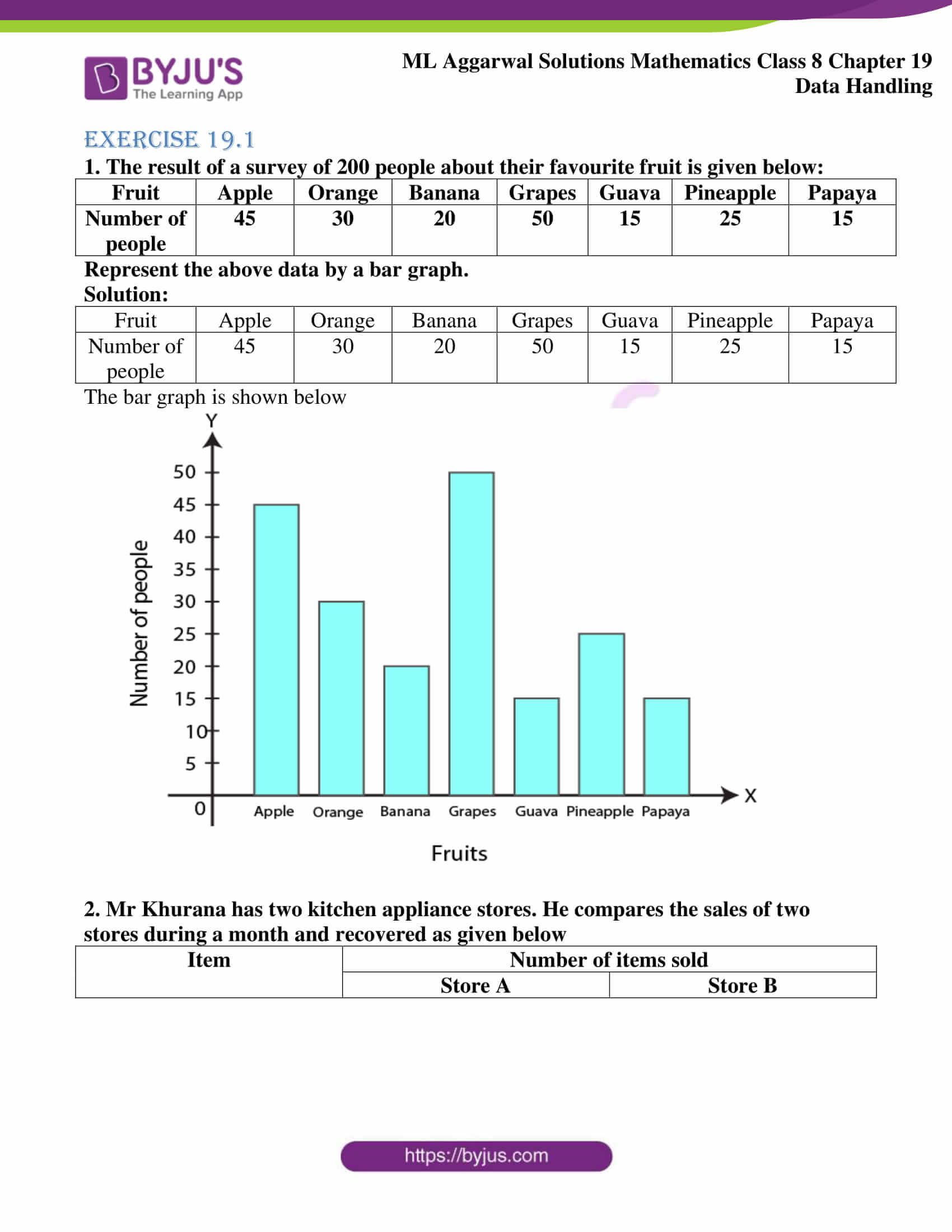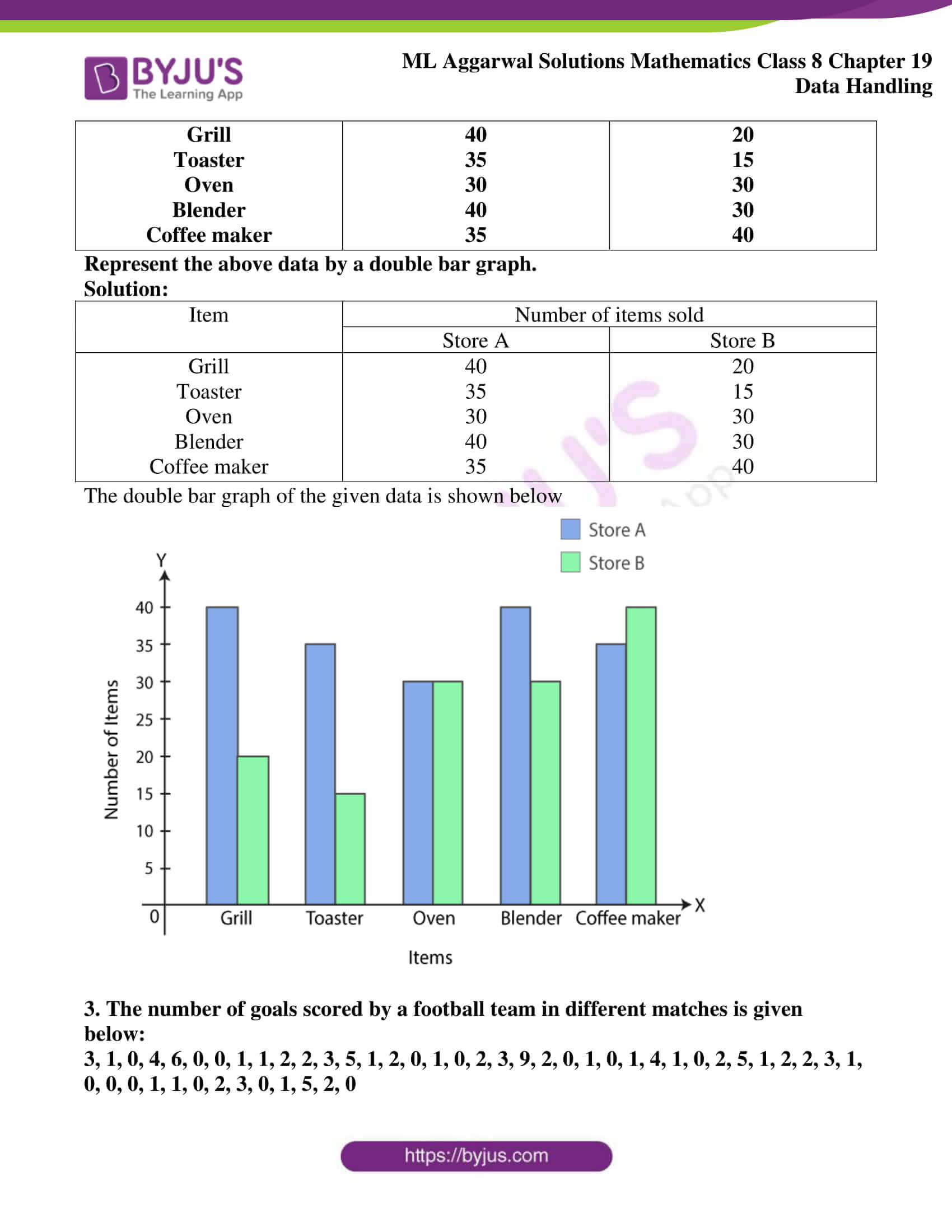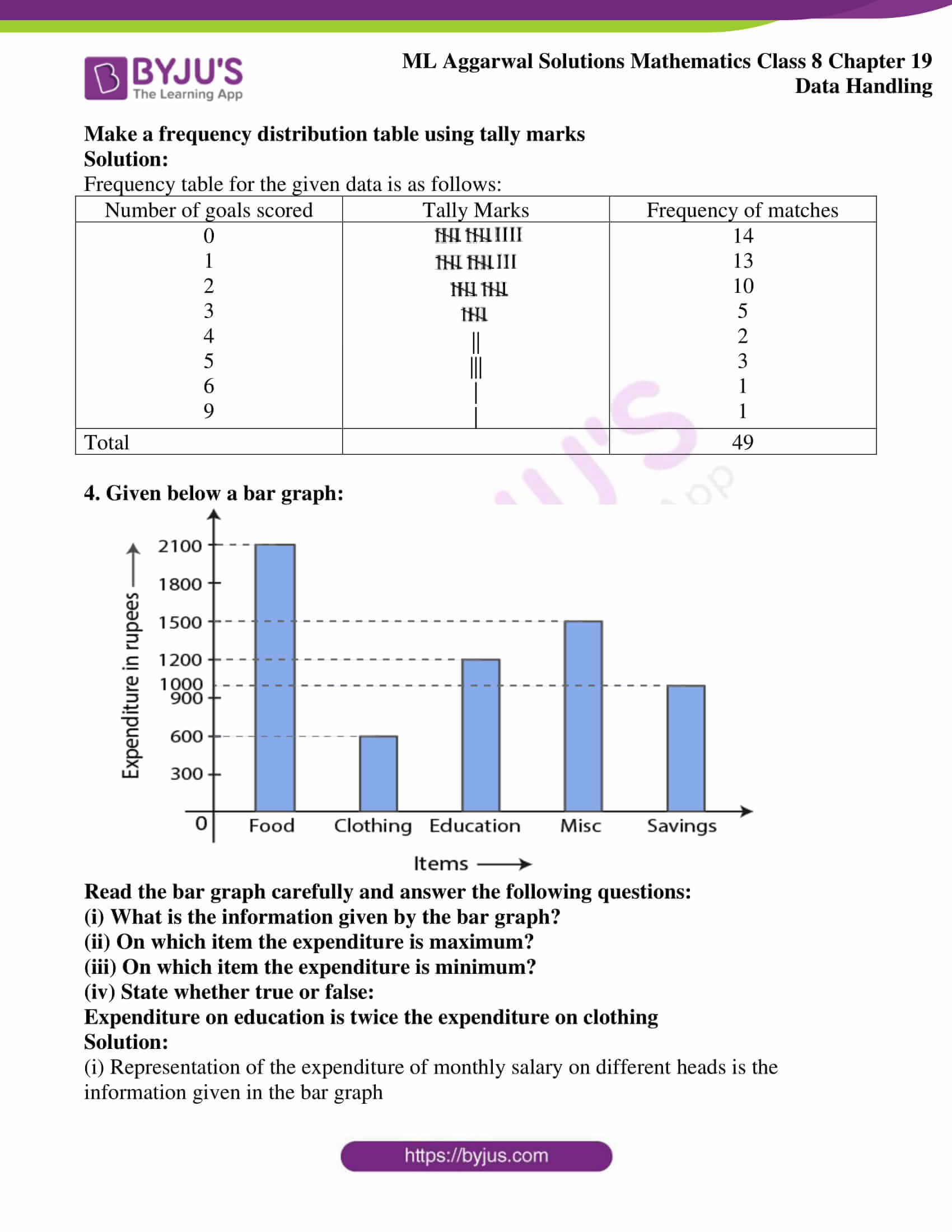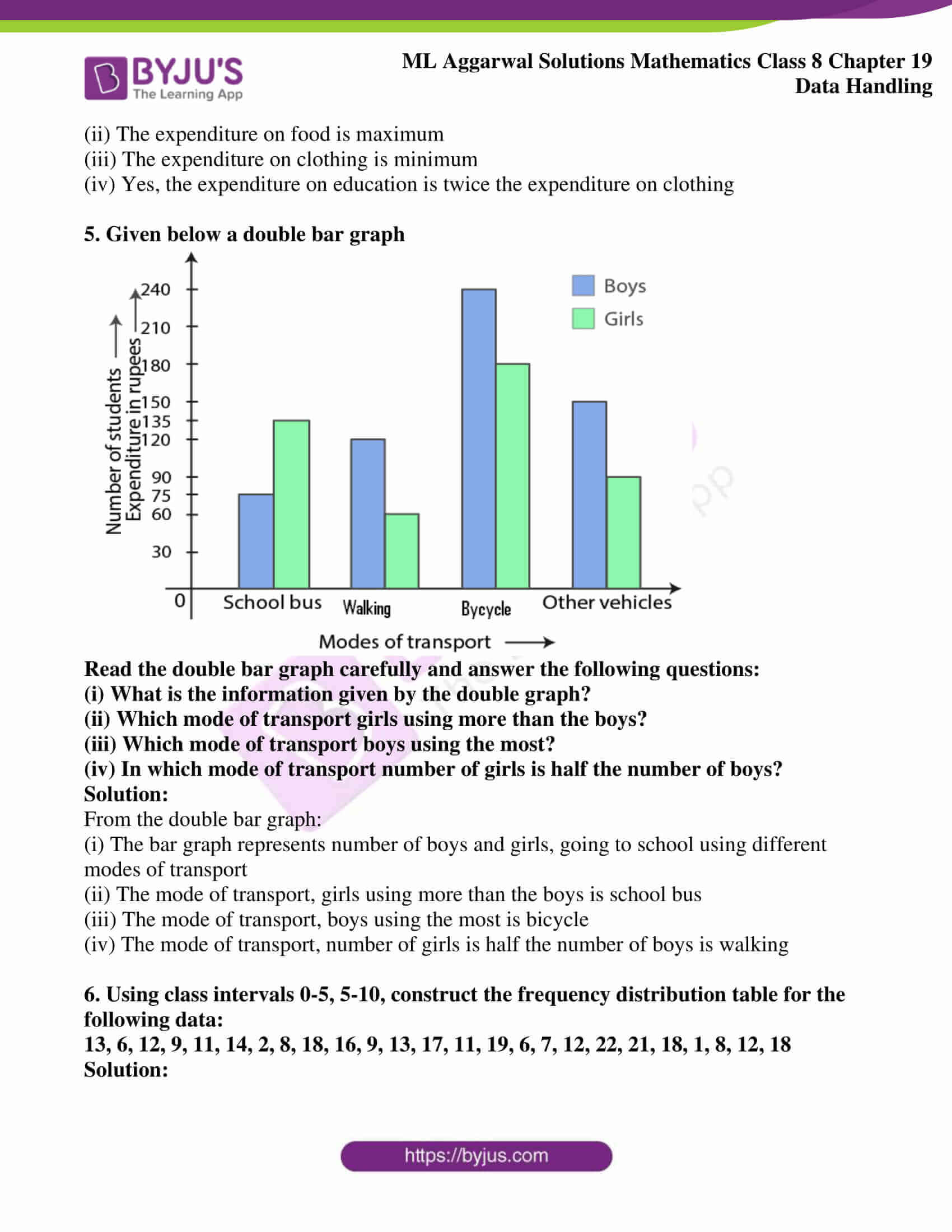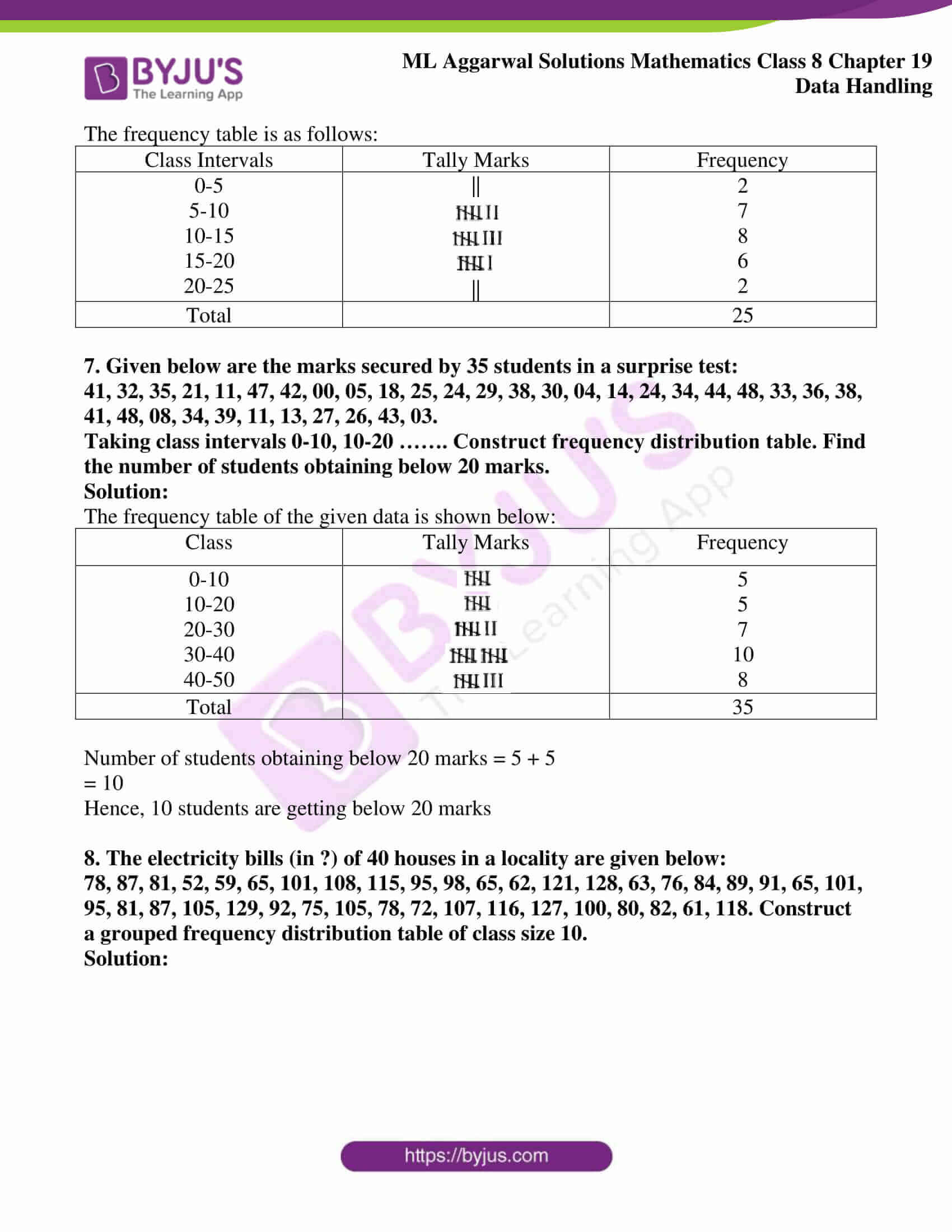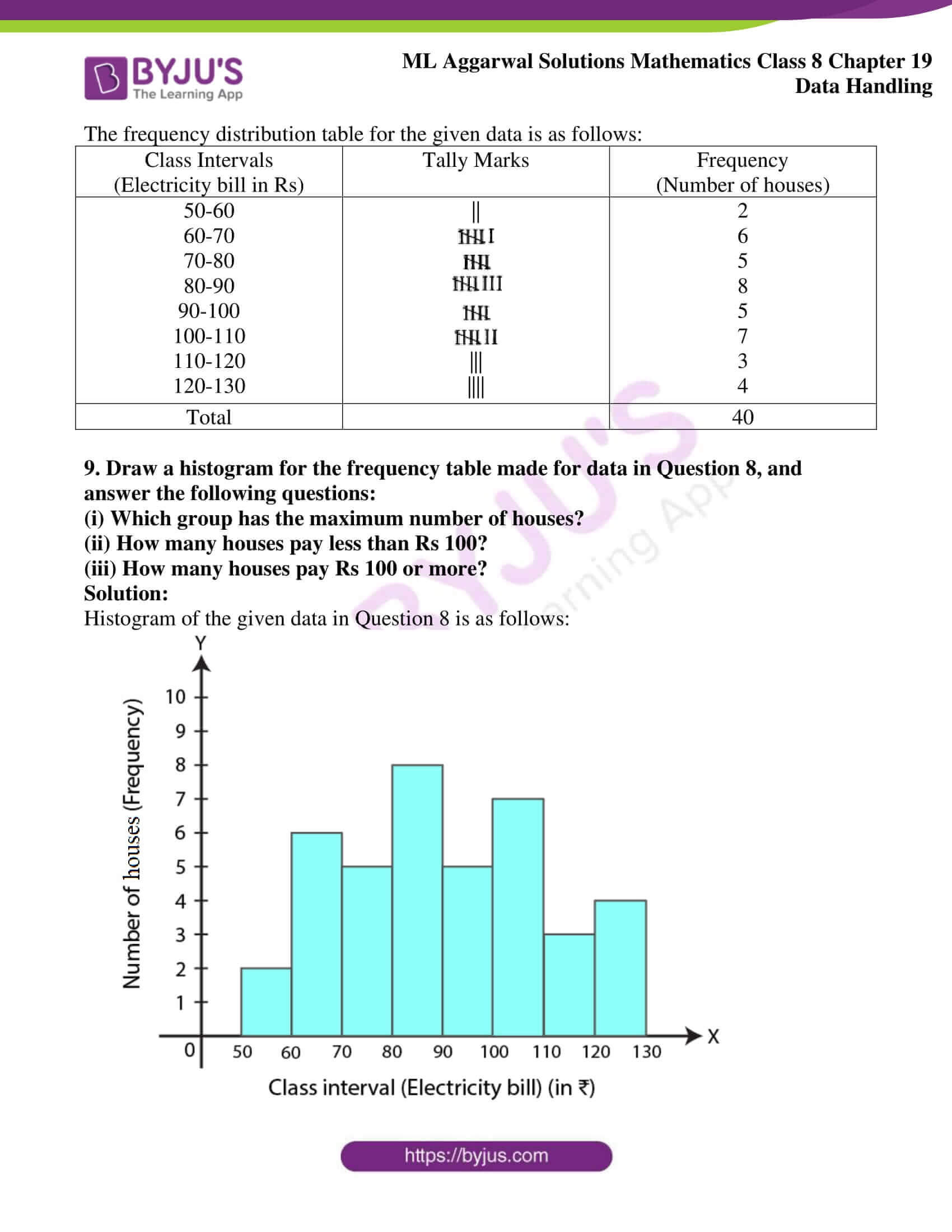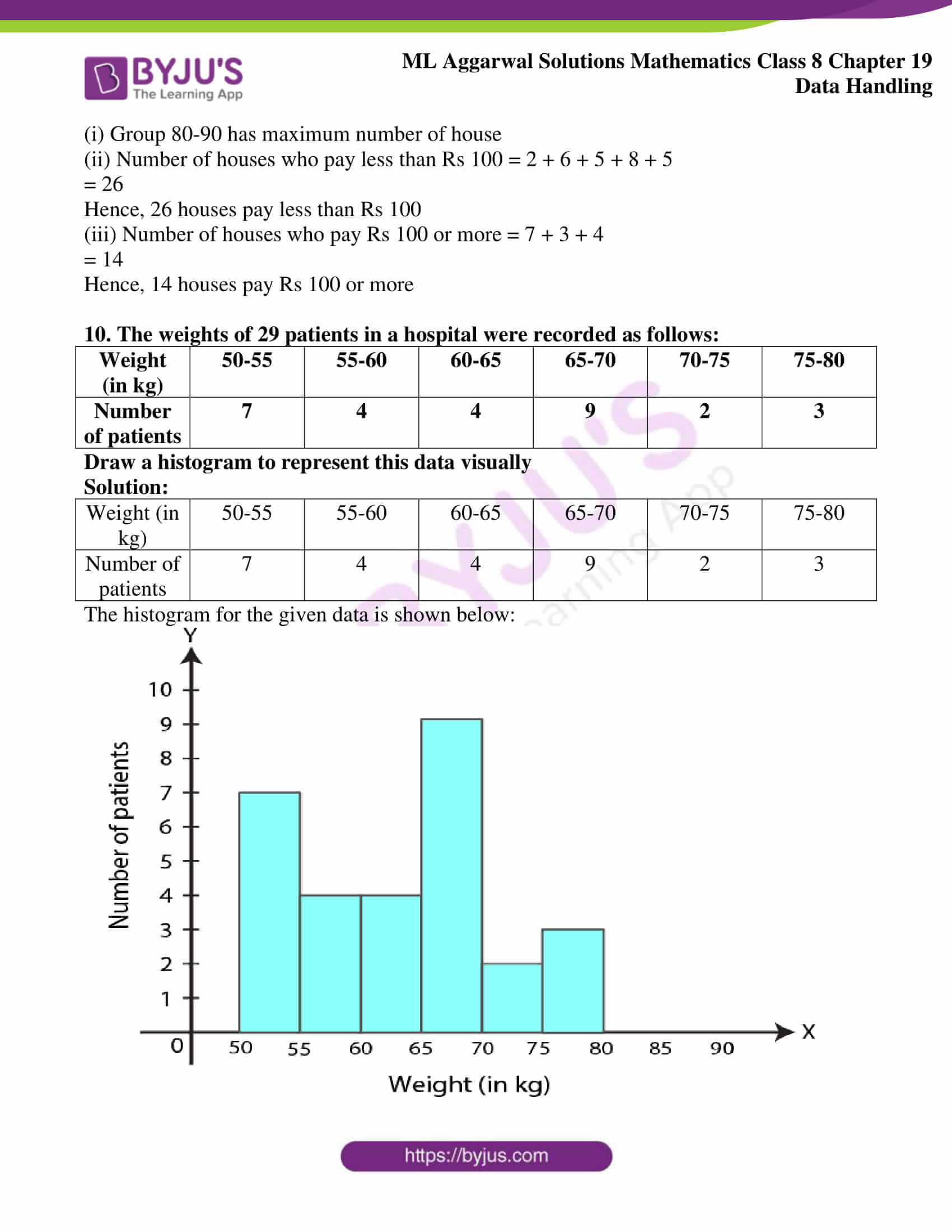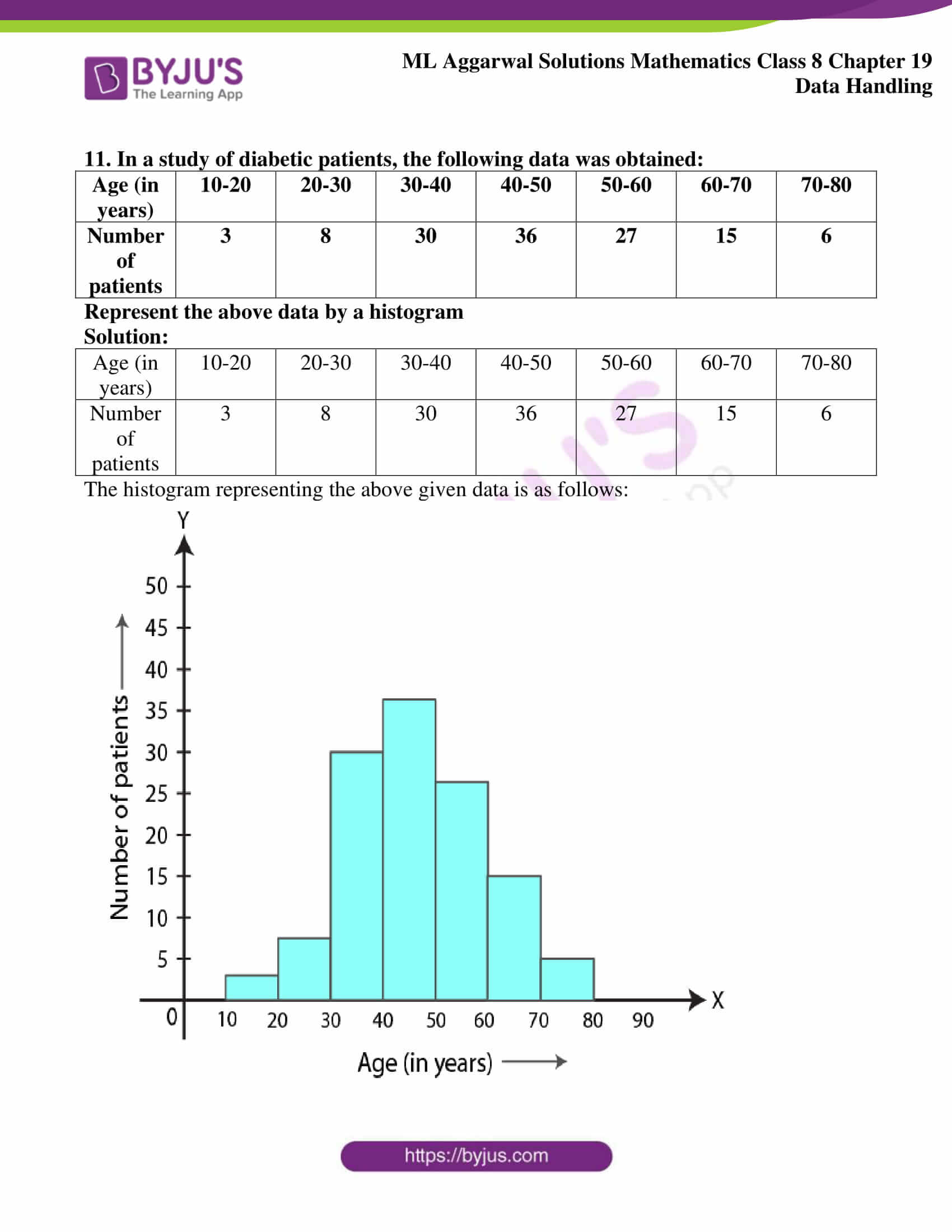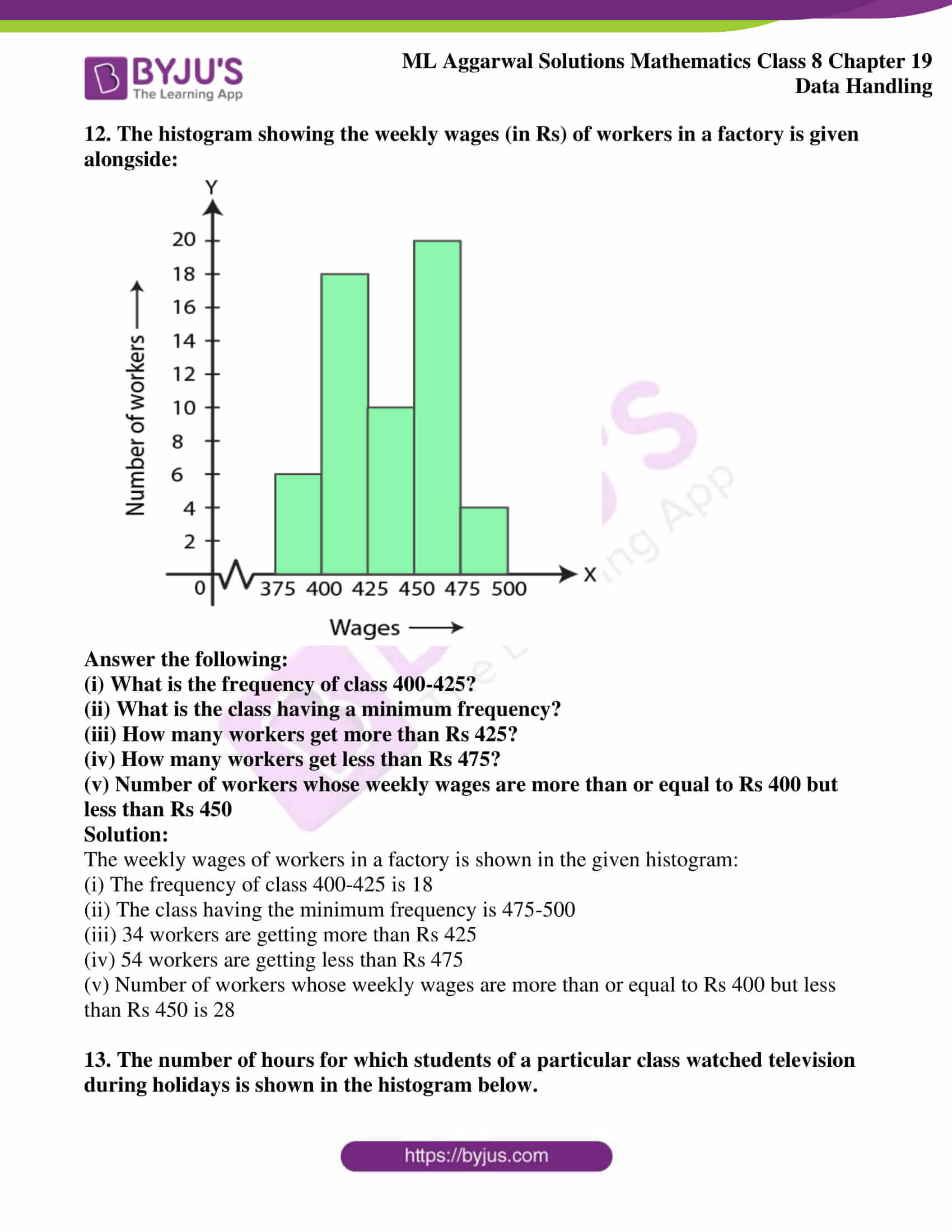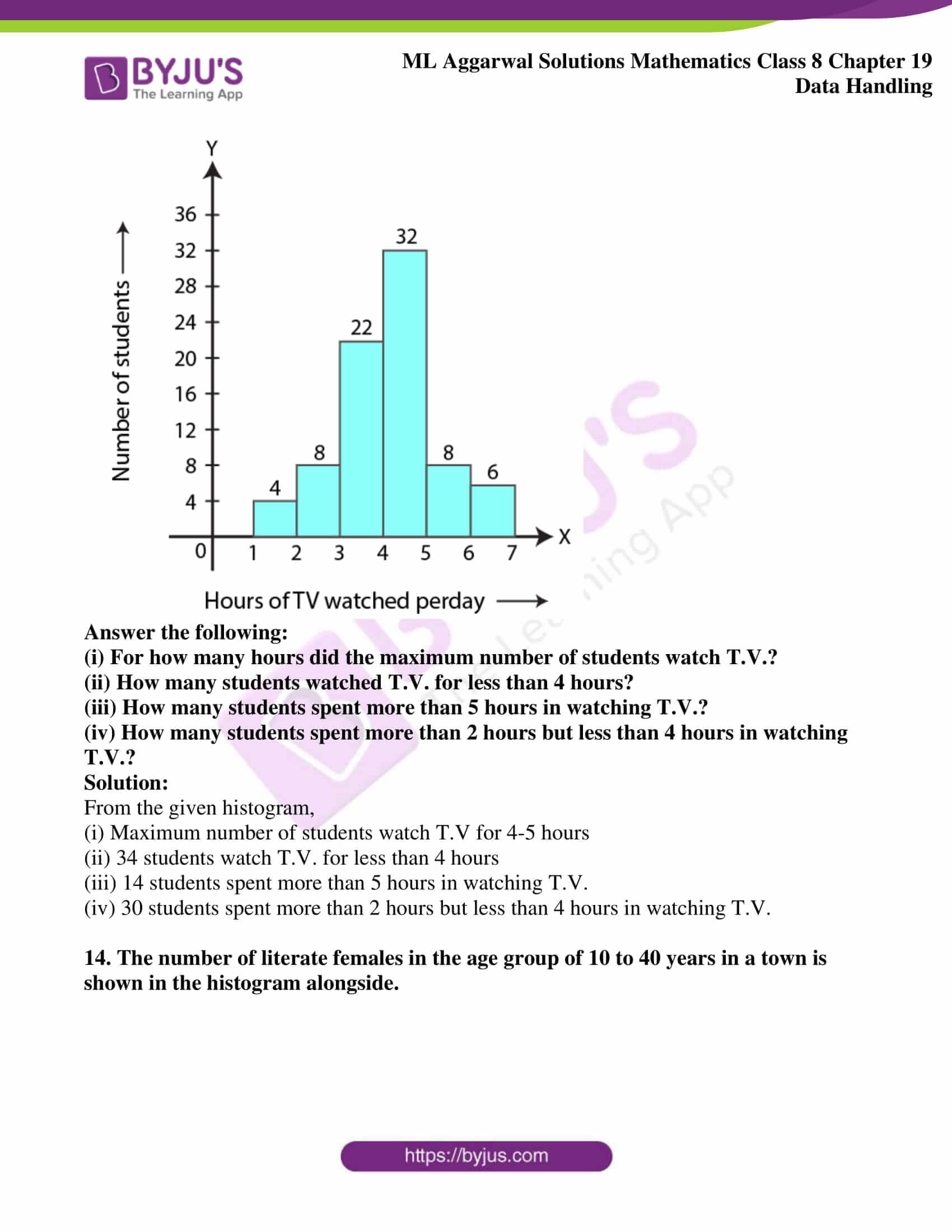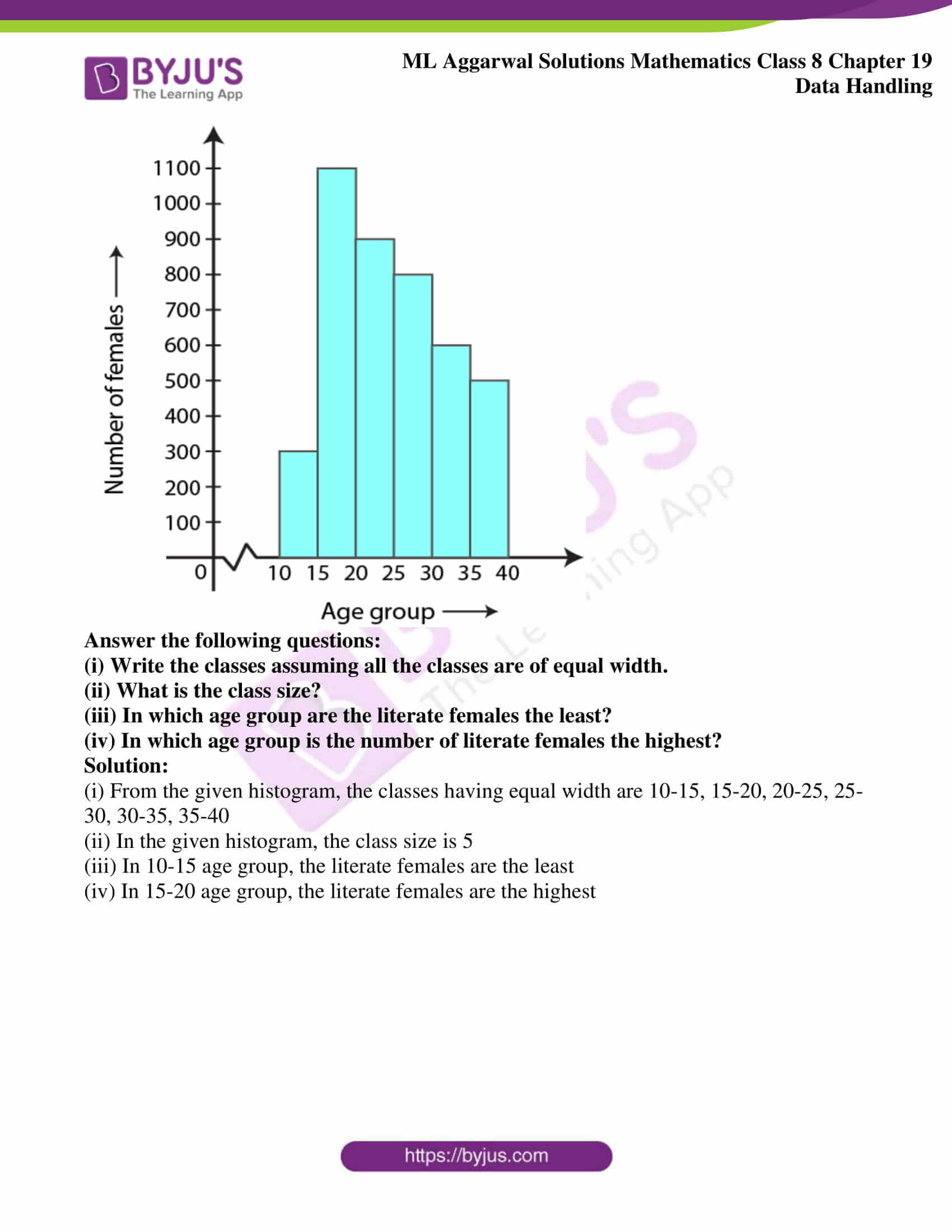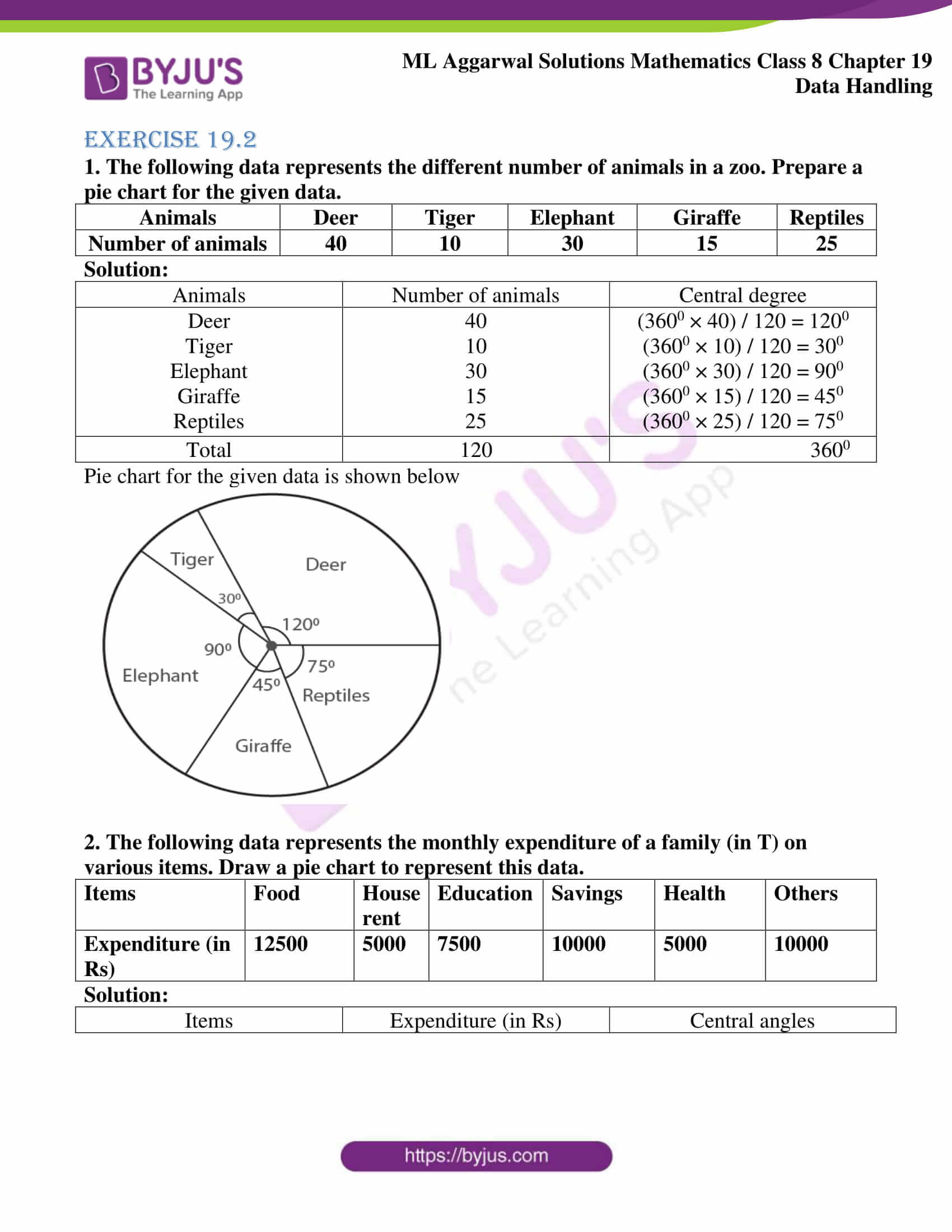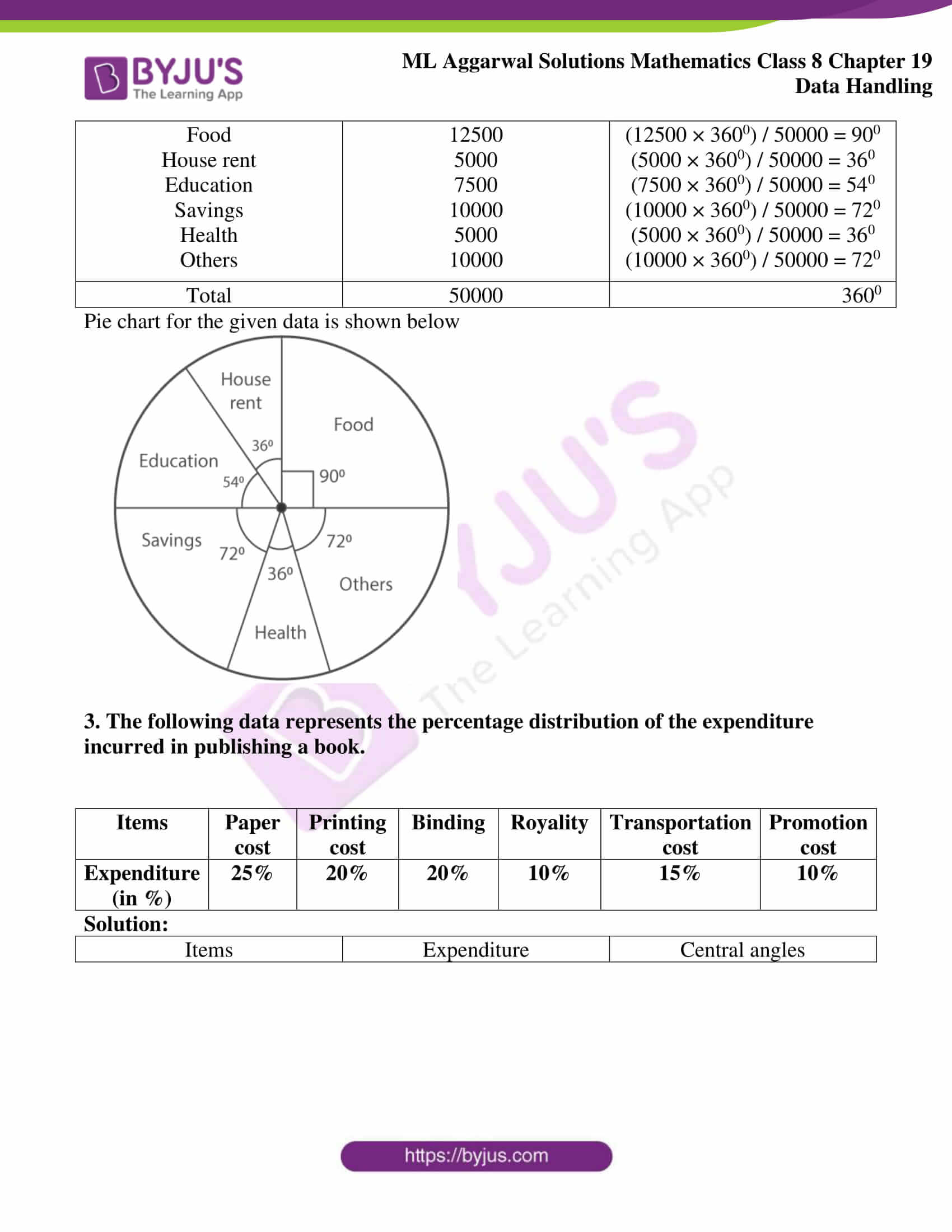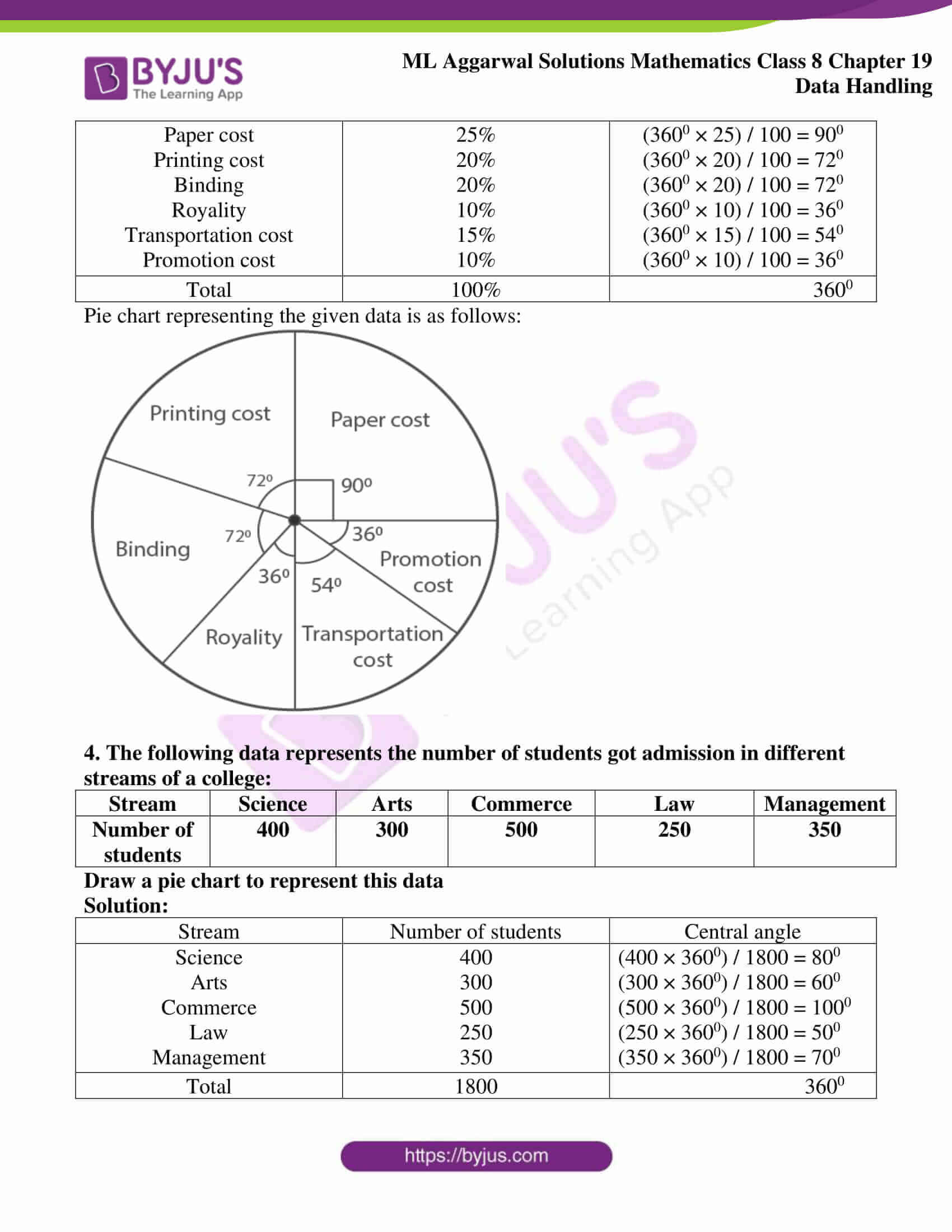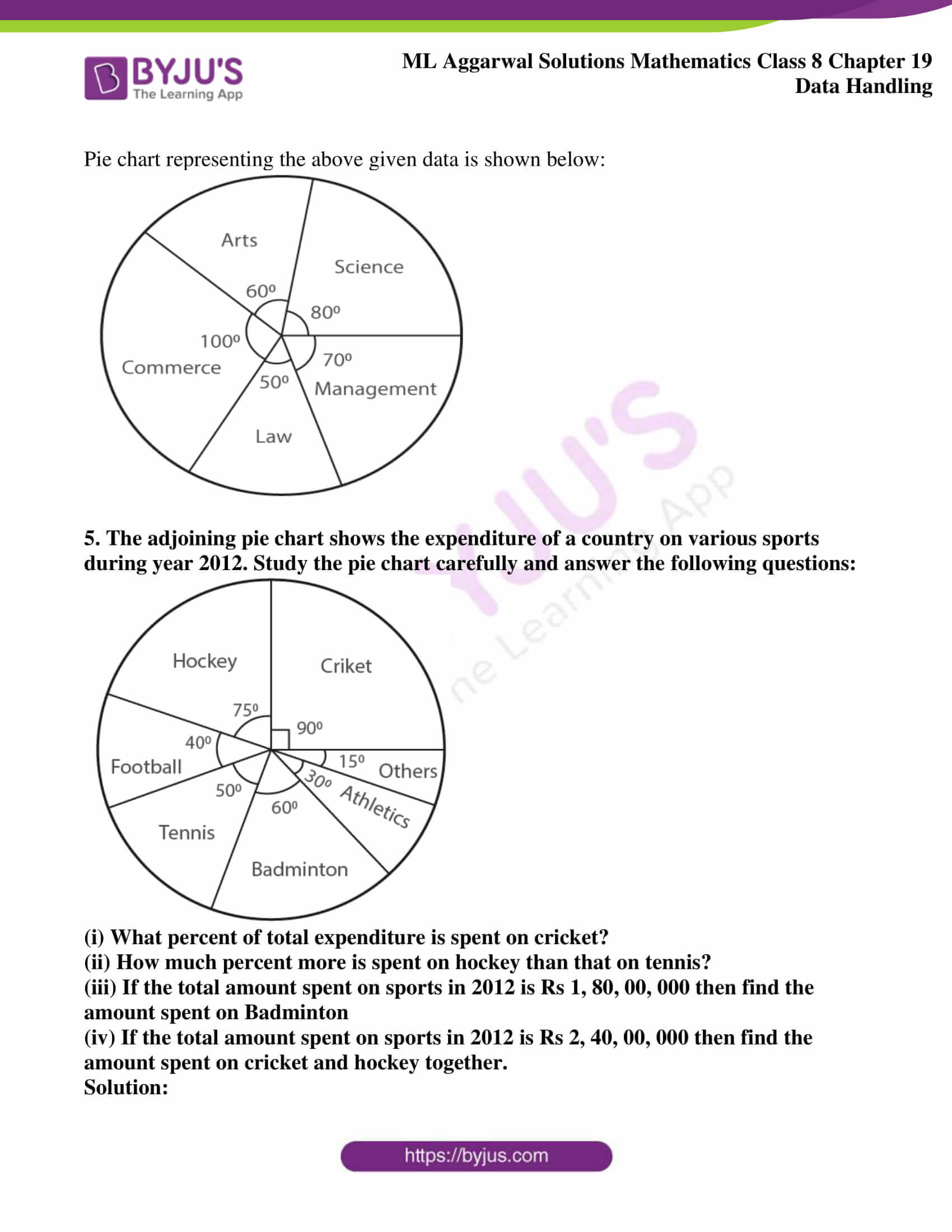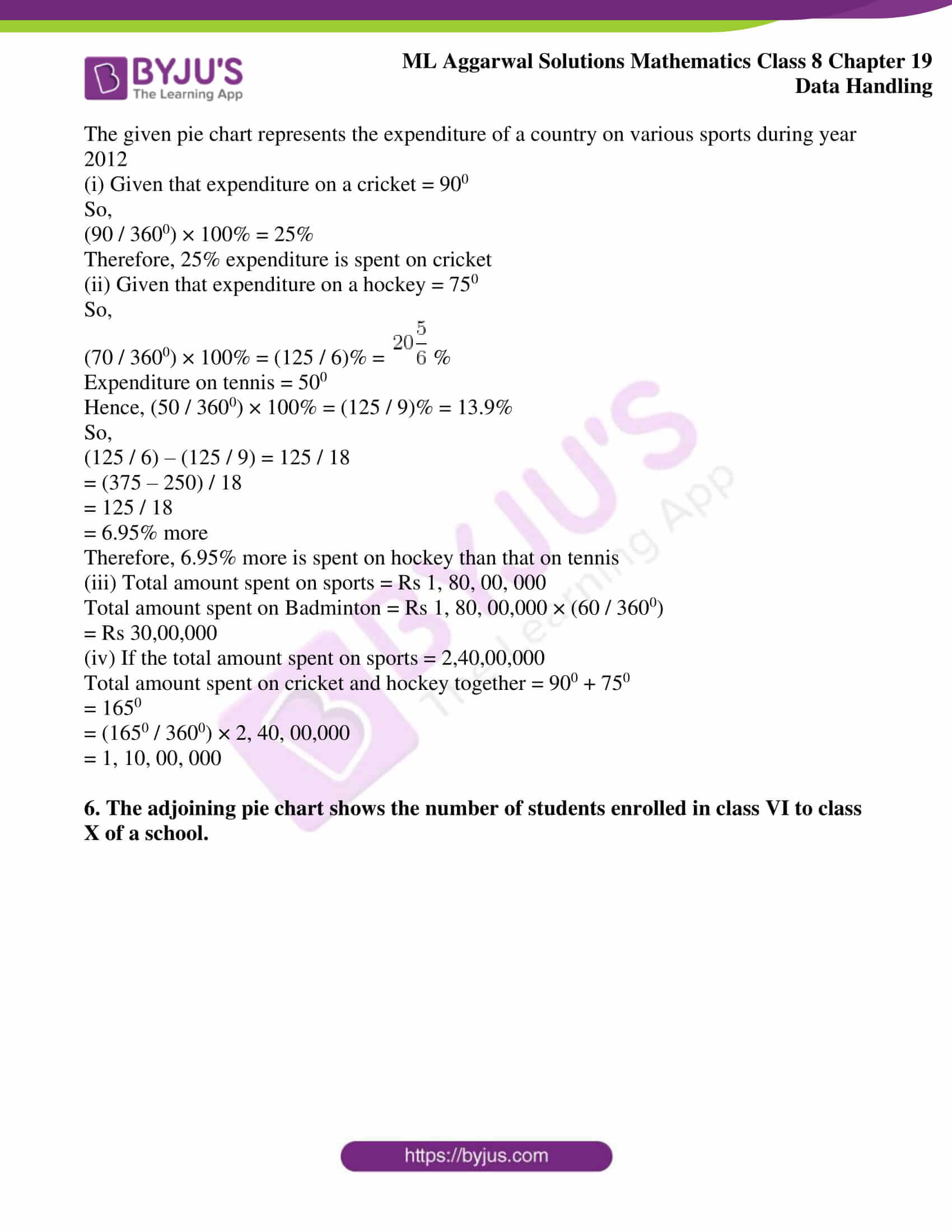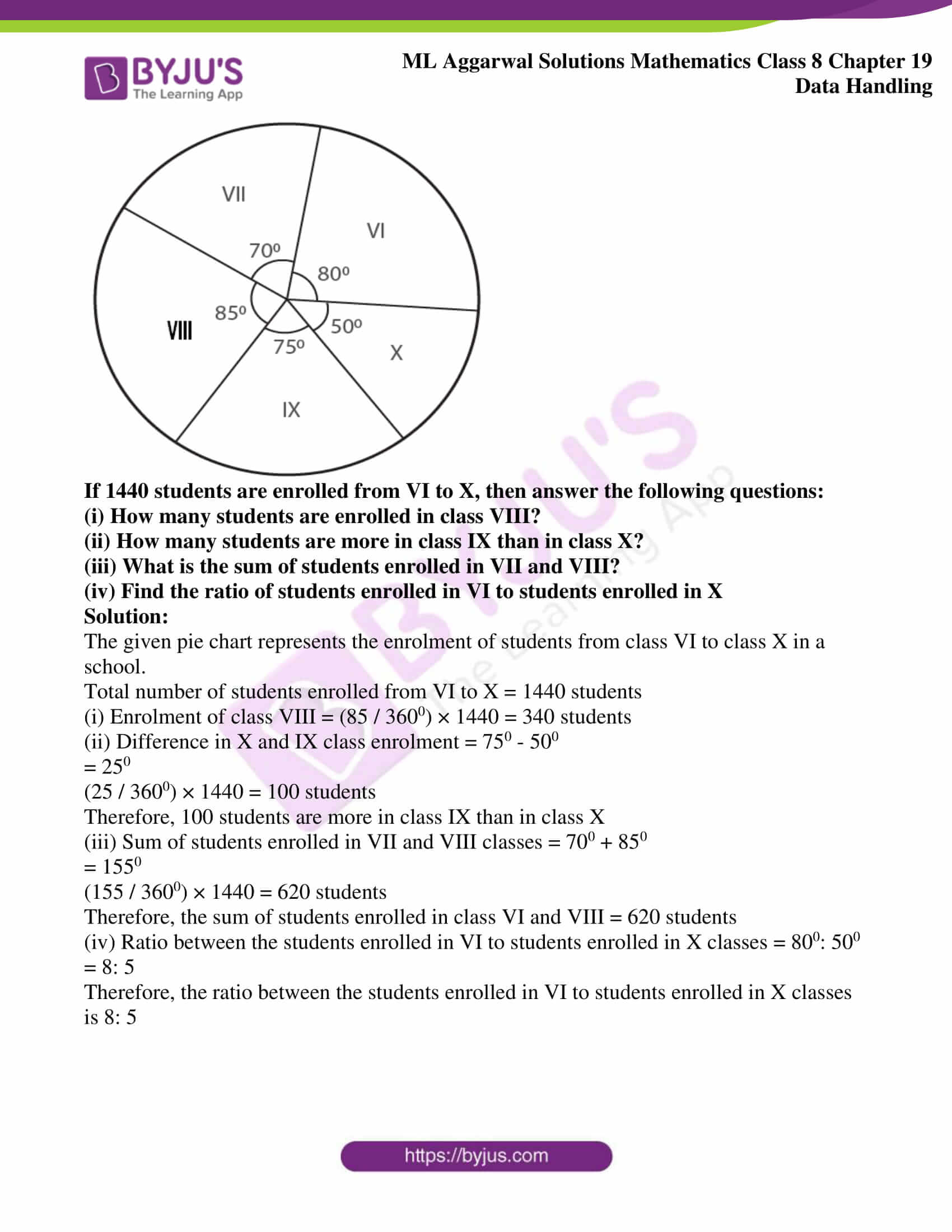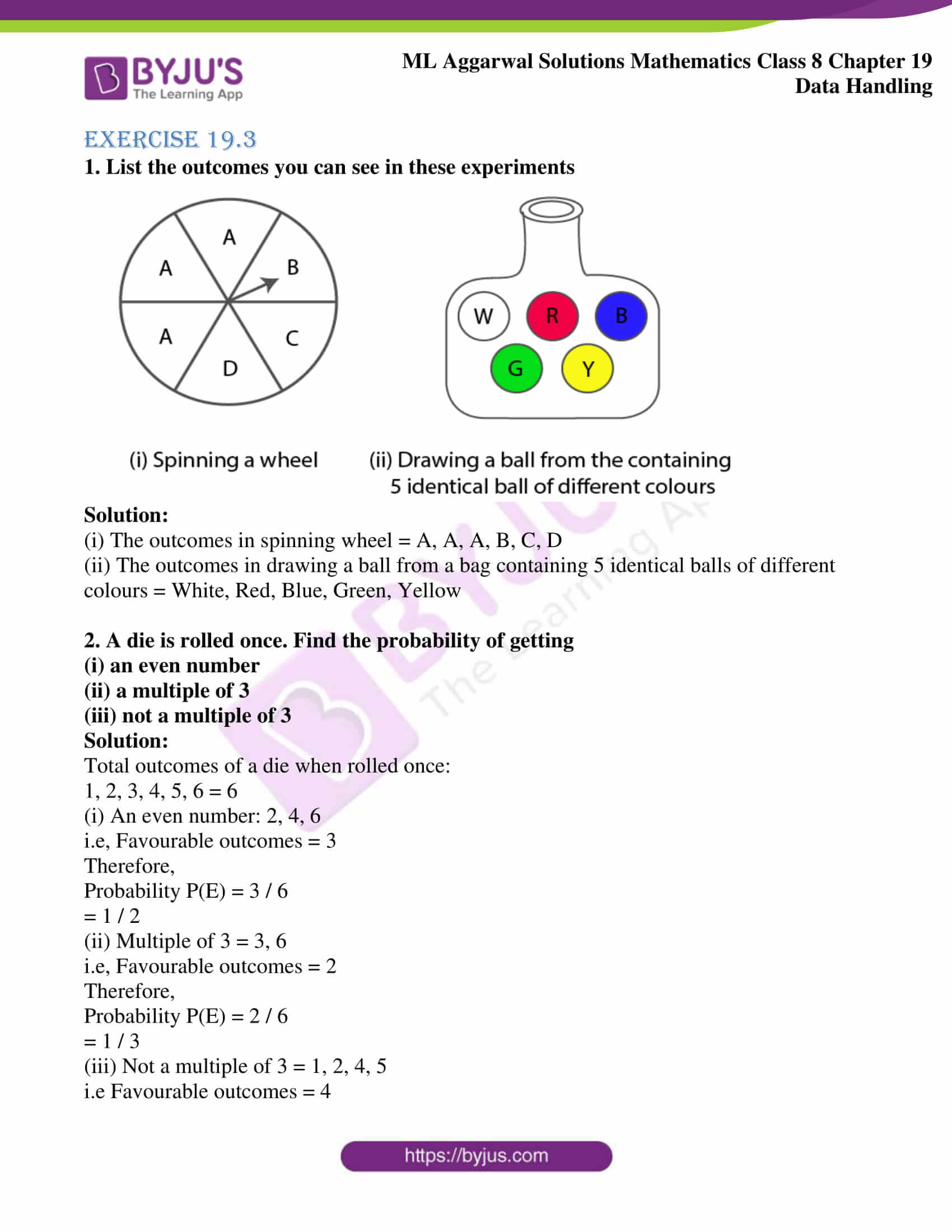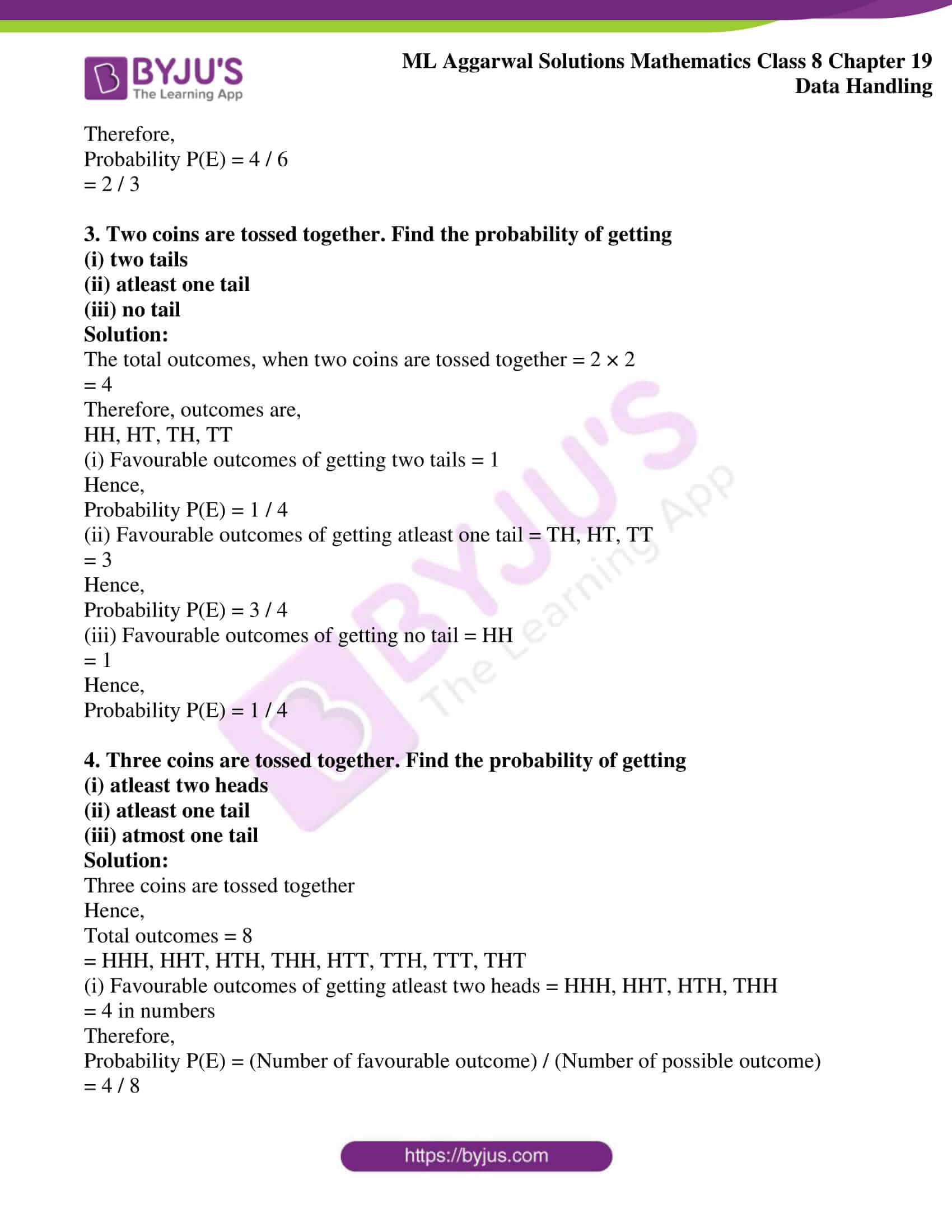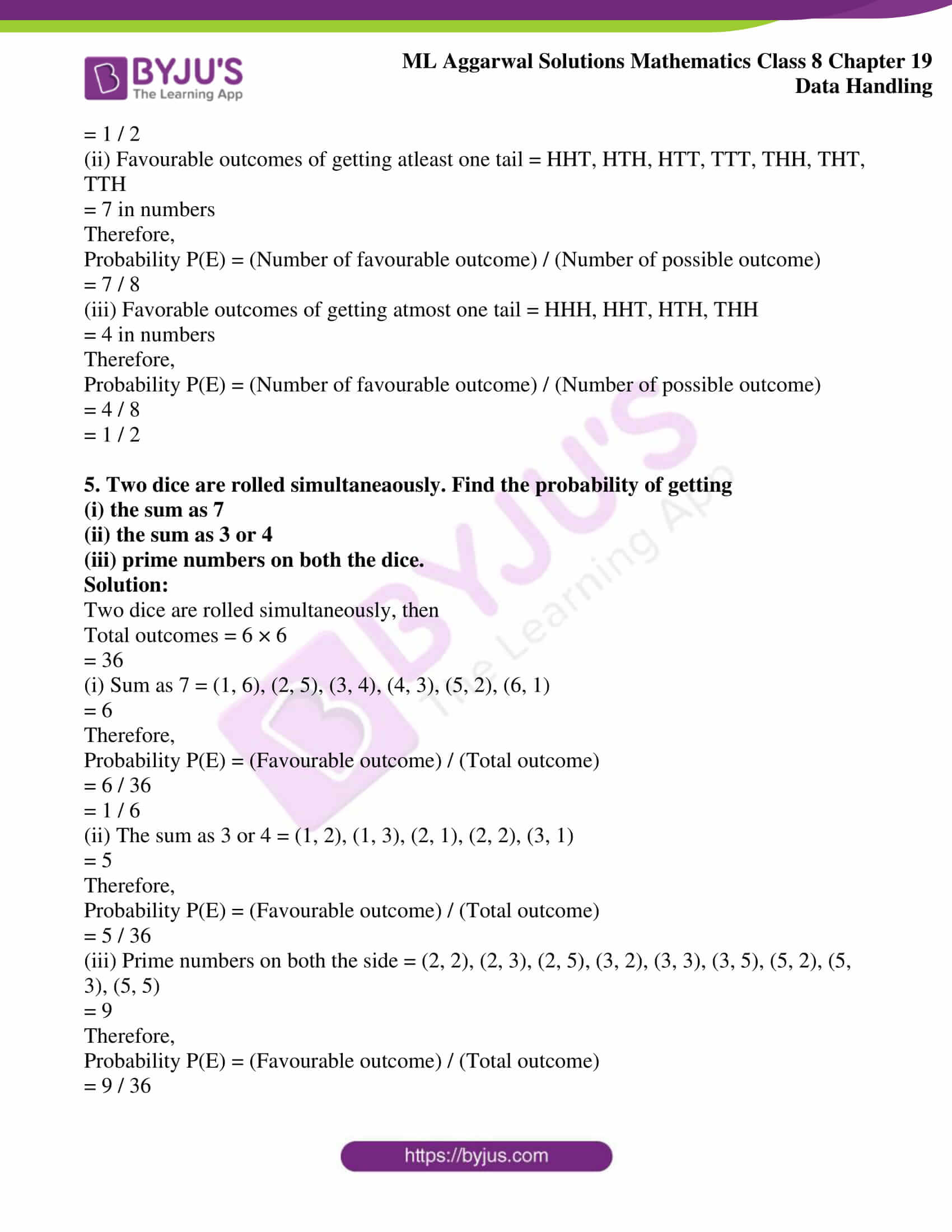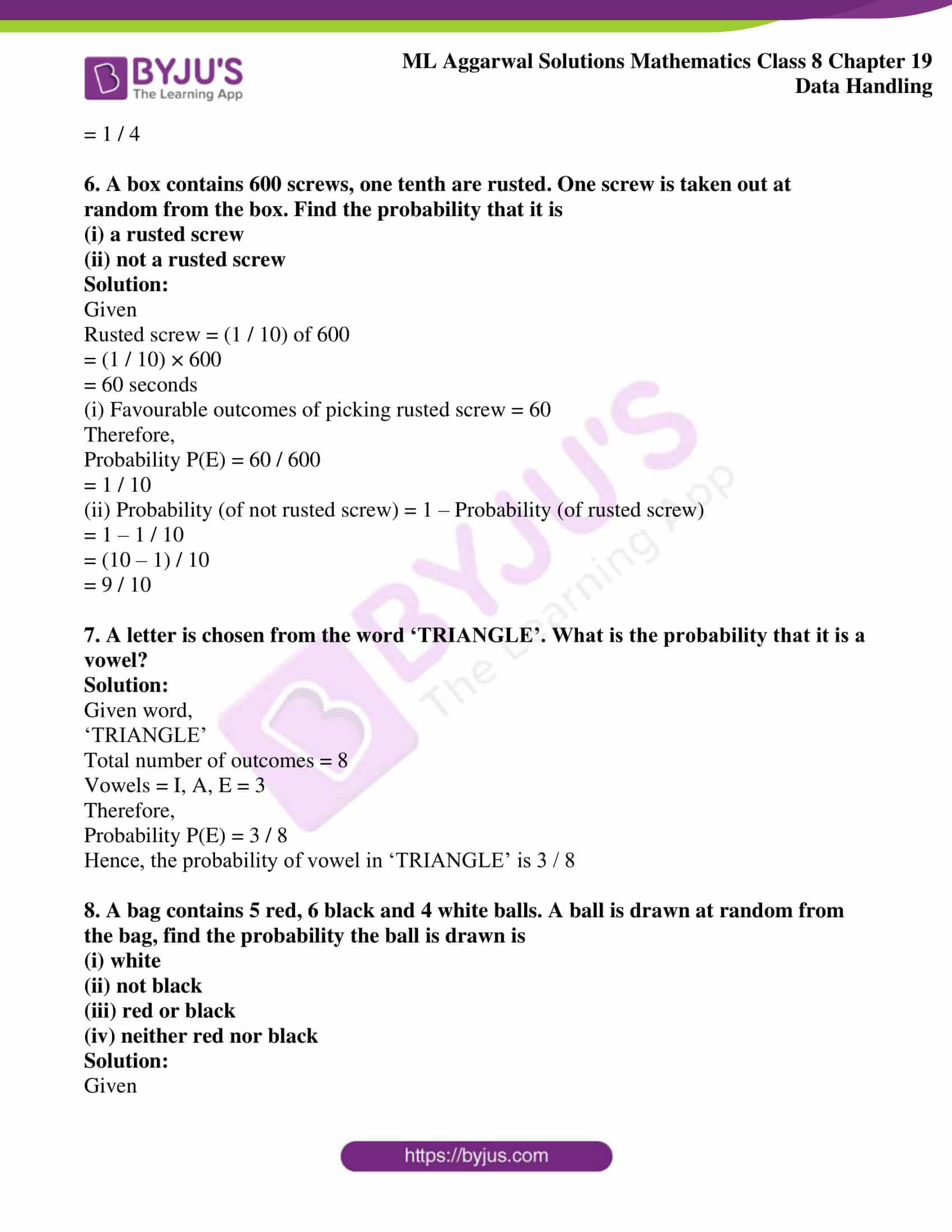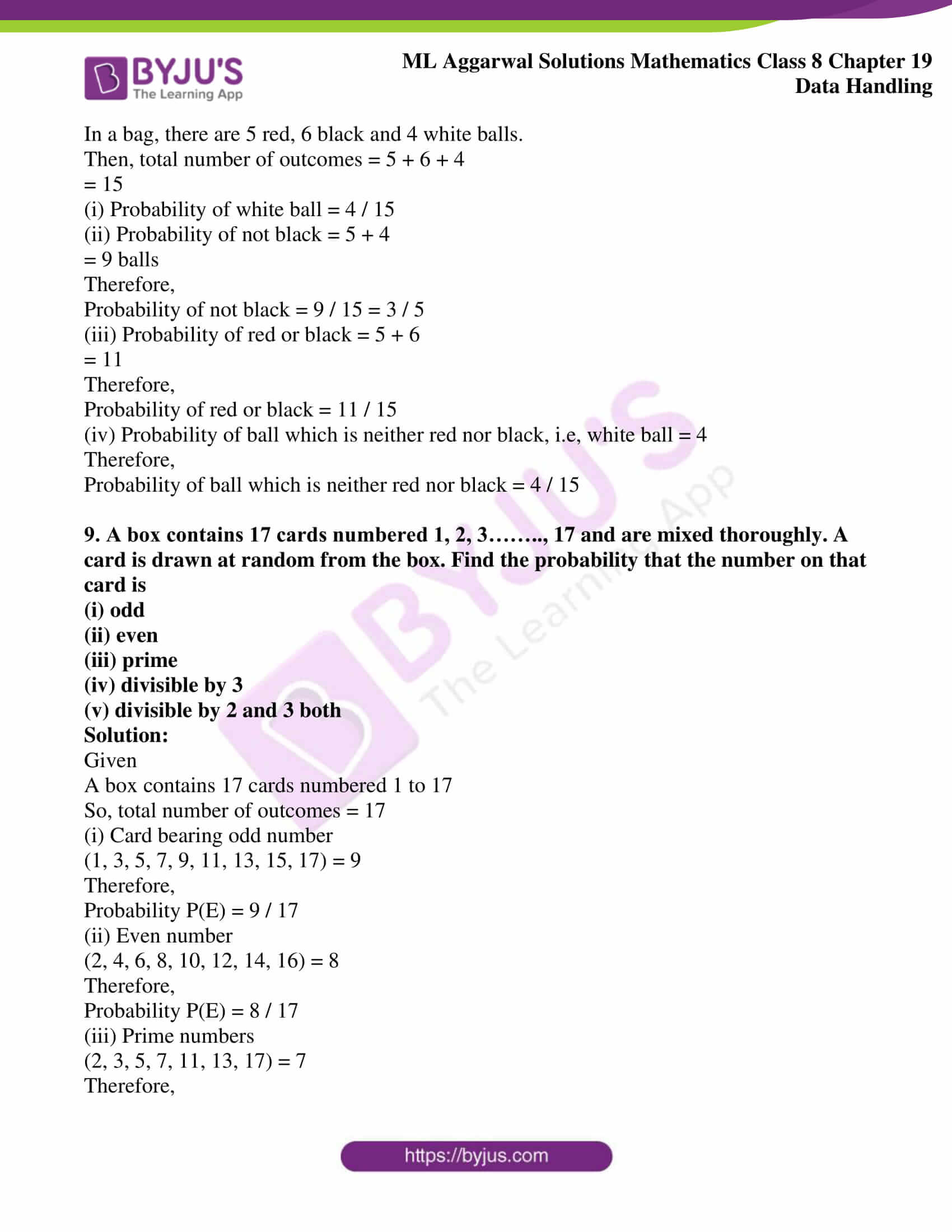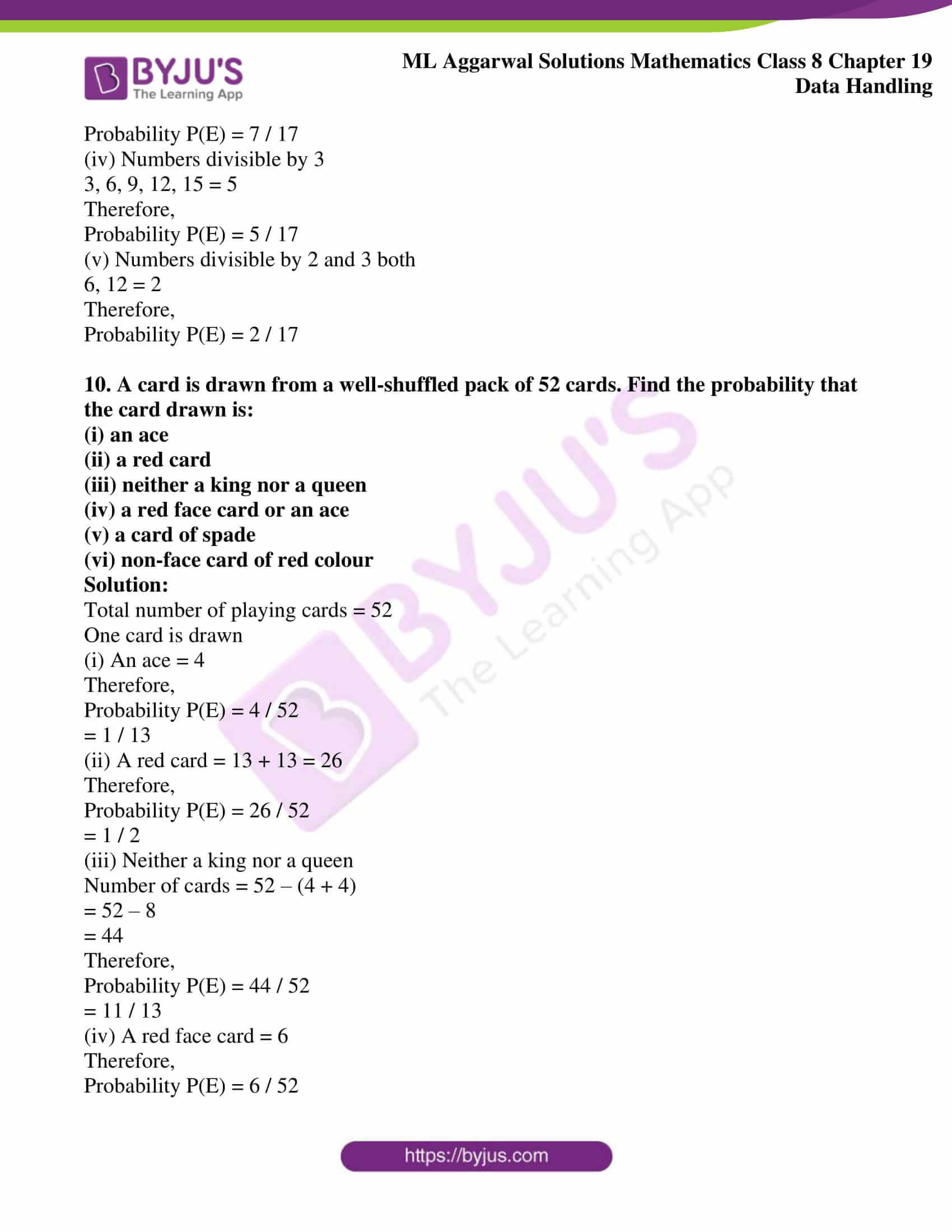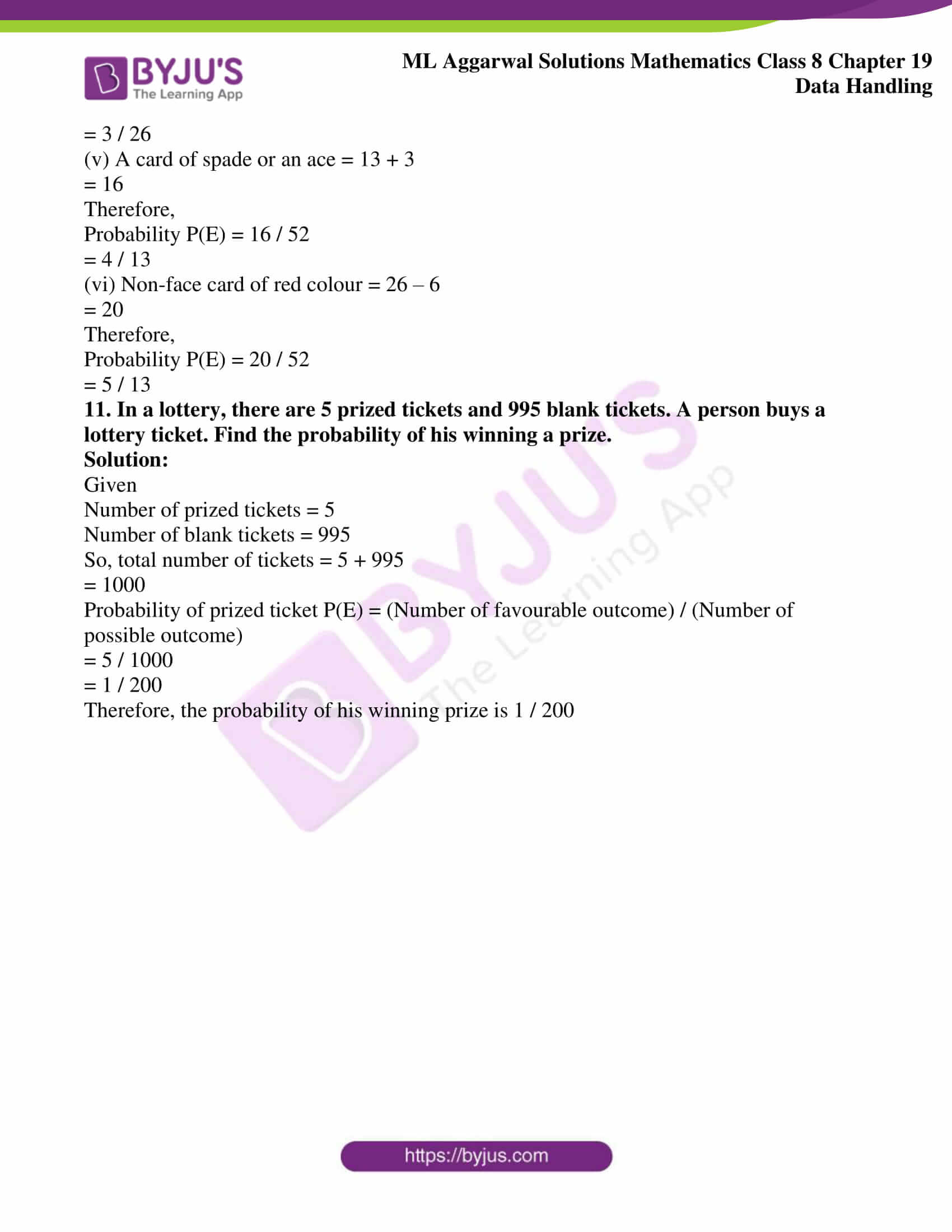## Access ML Aggarwal Solutions for Class 8 Maths Chapter 19 Data Handling

Exercise 19.1

1. The result of a survey of 200 people about their favourite fruit is given below:

 Fruit Apple Orange Banana Grapes Guava Pineapple Papaya Number of people 45 30 20 50 15 25 15

Represent the above data by a bar graph.

Solution:

 Fruit Apple Orange Banana Grapes Guava Pineapple Papaya Number of people 45 30 20 50 15 25 15

The bar graph is shown below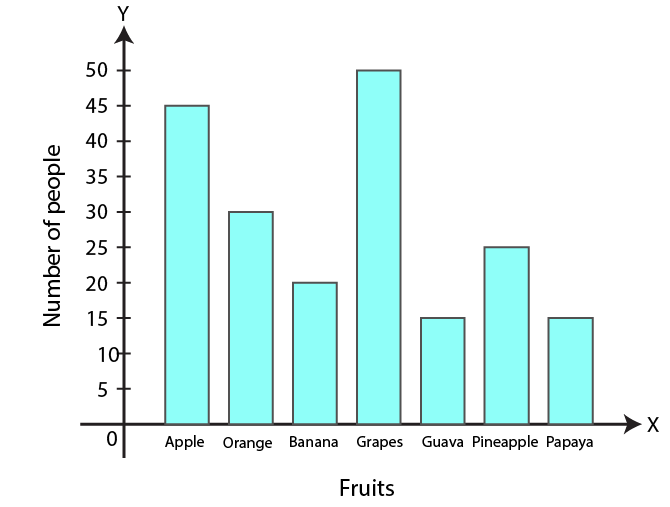2. Mr Khurana has two kitchen appliance stores. He compares the sales of two stores during a month and recovered as given below

 Item Number of items sold Store A Store B Grill Toaster Oven Blender Coffee maker 40 35 30 40 35 20 15 30 30 40

Represent the above data by a double bar graph.

Solution:

 Item Number of items sold Store A Store B Grill Toaster Oven Blender Coffee maker 40 35 30 40 35 20 15 30 30 40

The double bar graph of the given data is shown below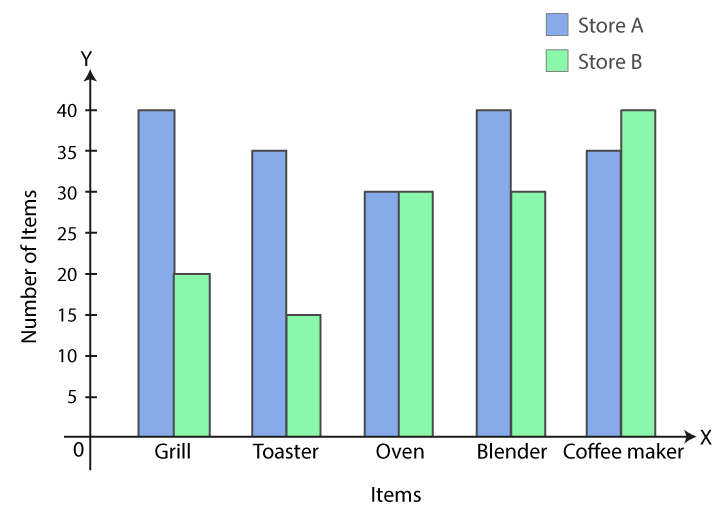3. The number of goals scored by a football team in different matches is given below:

3, 1, 0, 4, 6, 0, 0, 1, 1, 2, 2, 3, 5, 1, 2, 0, 1, 0, 2, 3, 9, 2, 0, 1, 0, 1, 4, 1, 0, 2, 5, 1, 2, 2, 3, 1, 0, 0, 0, 1, 1, 0, 2, 3, 0, 1, 5, 2, 0

Make a frequency distribution table using tally marks

Solution:

Frequency table for the given data is as follows:

 Number of goals scored Tally Marks Frequency of matches 0 1 2 3 4 5 6 9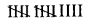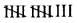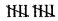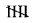|| ||| | | 14 13 10 5 2 3 1 1 Total 49

4. Given below a bar graph: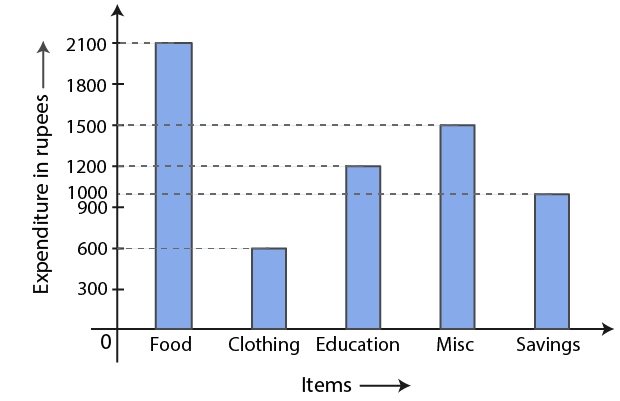(i) What is the information given by the bar graph?

(ii) On which item the expenditure is maximum?

(iii) On which item the expenditure is minimum?

(iv) State whether true or false:

Expenditure on education is twice the expenditure on clothing

Solution:

(i) Representation of the expenditure of monthly salary on different heads is the information given in the bar graph

(ii) The expenditure on food is maximum

(iii) The expenditure on clothing is minimum

(iv) Yes, the expenditure on education is twice the expenditure on clothing

5. Given below a double bar graph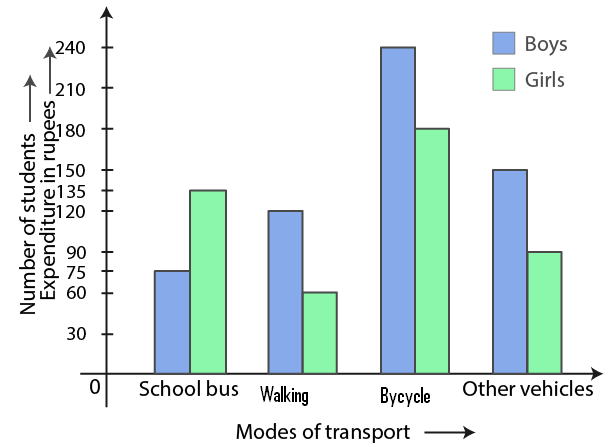(i) What is the information given by the double graph?

(ii) Which mode of transport girls using more than the boys?

(iii) Which mode of transport boys using the most?

(iv) In which mode of transport number of girls is half the number of boys?

Solution:

From the double bar graph:

(i) The bar graph represents number of boys and girls, going to school using different modes of transport

(ii) The mode of transport, girls using more than the boys is school bus

(iii) The mode of transport, boys using the most is bicycle

(iv) The mode of transport, number of girls is half the number of boys is walking

6. Using class intervals 0-5, 5-10, construct the frequency distribution table for the following data:

13, 6, 12, 9, 11, 14, 2, 8, 18, 16, 9, 13, 17, 11, 19, 6, 7, 12, 22, 21, 18, 1, 8, 12, 18

Solution:

The frequency table is as follows:

 Class Intervals Tally Marks Frequency 0-5 5-10 10-15 15-20 20-25 ||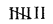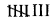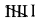|| 2 7 8 6 2 Total 25

7. Given below are the marks secured by 35 students in a surprise test:

41, 32, 35, 21, 11, 47, 42, 00, 05, 18, 25, 24, 29, 38, 30, 04, 14, 24, 34, 44, 48, 33, 36, 38, 41, 48, 08, 34, 39, 11, 13, 27, 26, 43, 03.

Taking class intervals 0-10, 10-20 ……. Construct frequency distribution table. Find the number of students obtaining below 20 marks.

Solution:

The frequency table of the given data is shown below:

 Class Tally Marks Frequency 0-10 10-20 20-30 30-40 40-50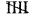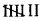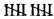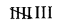5 5 7 10 8 Total 35

Number of students obtaining below 20 marks = 5 + 5

= 10

Hence, 10 students are getting below 20 marks

8. The electricity bills (in ?) of 40 houses in a locality are given below:

78, 87, 81, 52, 59, 65, 101, 108, 115, 95, 98, 65, 62, 121, 128, 63, 76, 84, 89, 91, 65, 101, 95, 81, 87, 105, 129, 92, 75, 105, 78, 72, 107, 116, 127, 100, 80, 82, 61, 118. Construct a grouped frequency distribution table of class size 10.

Solution:

The frequency distribution table for the given data is as follows:

 Class Intervals (Electricity bill in Rs) Tally Marks Frequency (Number of houses) 50-60 60-70 70-80 80-90 90-100 100-110 110-120 120-130 ||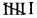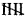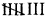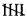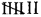||| |||| 2 6 5 8 5 7 3 4 Total 40

9. Draw a histogram for the frequency table made for data in Question 8, and answer the following questions:

(i) Which group has the maximum number of houses?

(ii) How many houses pay less than Rs 100?

(iii) How many houses pay Rs 100 or more?

Solution:

Histogram of the given data in Question 8 is as follows: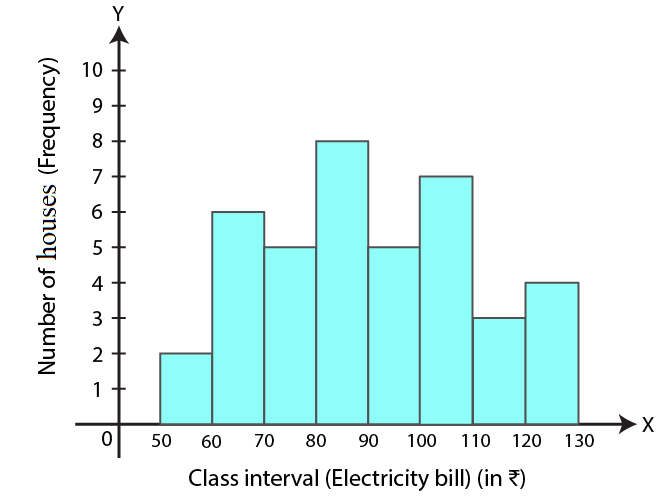(i) Group 80-90 has maximum number of house

(ii) Number of houses who pay less than Rs 100 = 2 + 6 + 5 + 8 + 5

= 26

Hence, 26 houses pay less than Rs 100

(iii) Number of houses who pay Rs 100 or more = 7 + 3 + 4

= 14

Hence, 14 houses pay Rs 100 or more

10. The weights of 29 patients in a hospital were recorded as follows:

 Weight (in kg) 50-55 55-60 60-65 65-70 70-75 75-80 Number of patients 7 4 4 9 2 3

Draw a histogram to represent this data visually

Solution:

 Weight (in kg) 50-55 55-60 60-65 65-70 70-75 75-80 Number of patients 7 4 4 9 2 3

The histogram for the given data is shown below: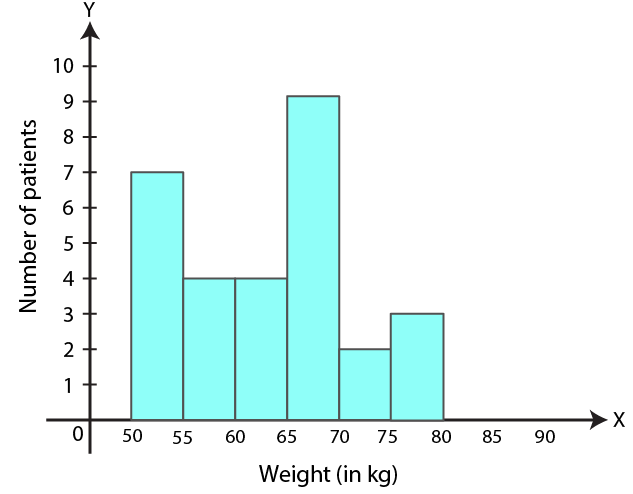11. In a study of diabetic patients, the following data was obtained:

 Age (in years) 10-20 20-30 30-40 40-50 50-60 60-70 70-80 Number of patients 3 8 30 36 27 15 6

Represent the above data by a histogram

Solution:

 Age (in years) 10-20 20-30 30-40 40-50 50-60 60-70 70-80 Number of patients 3 8 30 36 27 15 6

The histogram representing the above given data is as follows: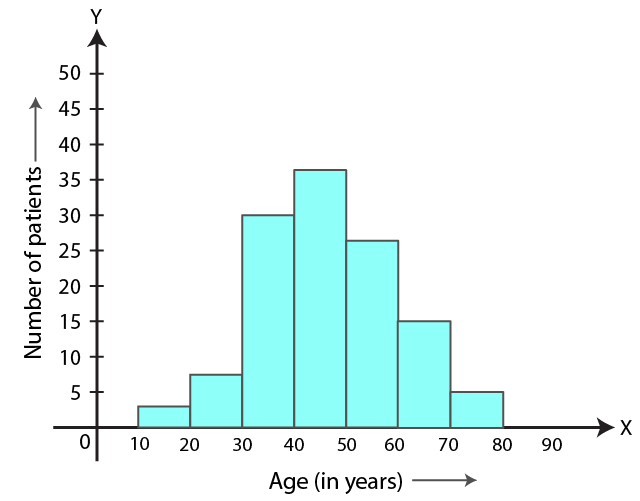12. The histogram showing the weekly wages (in Rs) of workers in a factory is given alongside: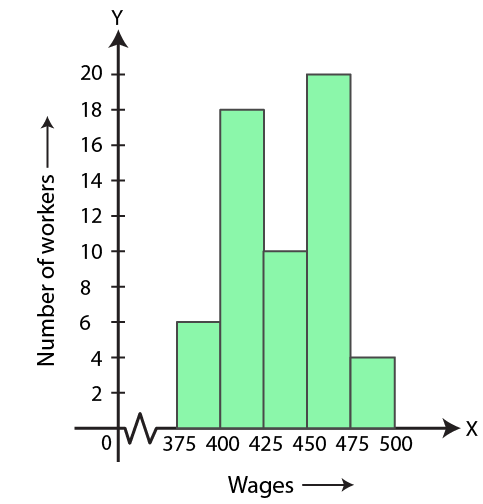(i) What is the frequency of class 400-425?

(ii) What is the class having a minimum frequency?

(iii) How many workers get more than Rs 425?

(iv) How many workers get less than Rs 475?

(v) Number of workers whose weekly wages are more than or equal to Rs 400 but less than Rs 450

Solution:

The weekly wages of workers in a factory is shown in the given histogram:

(i) The frequency of class 400-425 is 18

(ii) The class having the minimum frequency is 475-500

(iii) 34 workers are getting more than Rs 425

(iv) 54 workers are getting less than Rs 475

(v) Number of workers whose weekly wages are more than or equal to Rs 400 but less than Rs 450 is 28

13. The number of hours for which students of a particular class watched television during holidays is shown in the histogram below.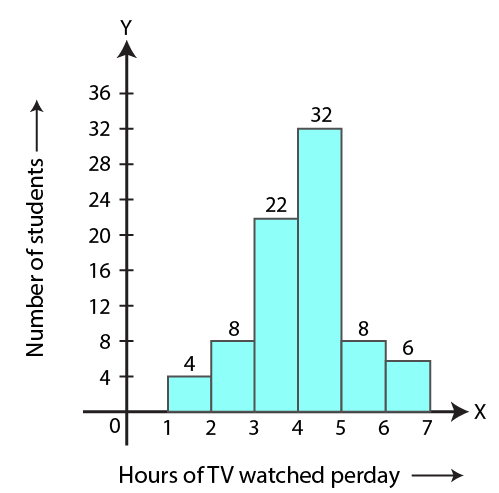(i) For how many hours did the maximum number of students watch T.V.?

(ii) How many students watched T.V. for less than 4 hours?

(iii) How many students spent more than 5 hours in watching T.V.?

(iv) How many students spent more than 2 hours but less than 4 hours in watching T.V.?

Solution:

From the given histogram,

(i) Maximum number of students watch T.V for 4-5 hours

(ii) 34 students watch T.V. for less than 4 hours

(iii) 14 students spent more than 5 hours in watching T.V.

(iv) 30 students spent more than 2 hours but less than 4 hours in watching T.V.

14. The number of literate females in the age group of 10 to 40 years in a town is shown in the histogram alongside.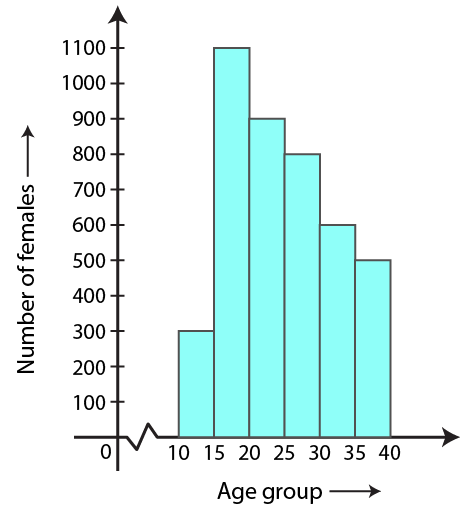(i) Write the classes assuming all the classes are of equal width.

(ii) What is the class size?

(iii) In which age group are the literate females the least?

(iv) In which age group is the number of literate females the highest?

Solution:

(i) From the given histogram, the classes having equal width are 10-15, 15-20, 20-25, 25-30, 30-35, 35-40

(ii) In the given histogram, the class size is 5

(iii) In 10-15 age group, the literate females are the least

(iv) In 15-20 age group, the literate females are the highest

Exercise 19.2

1. The following data represents the different number of animals in a zoo. Prepare a pie chart for the given data.

 Animals Deer Tiger Elephant Giraffe Reptiles Number of animals 40 10 30 15 25

Solution:

 Animals Number of animals Central degree Deer Tiger Elephant Giraffe Reptiles 40 10 30 15 25 (3600 × 40) / 120 = 1200 (3600 × 10) / 120 = 300 (3600 × 30) / 120 = 900 (3600 × 15) / 120 = 450 (3600 × 25) / 120 = 750 Total 120 3600

Pie chart for the given data is shown below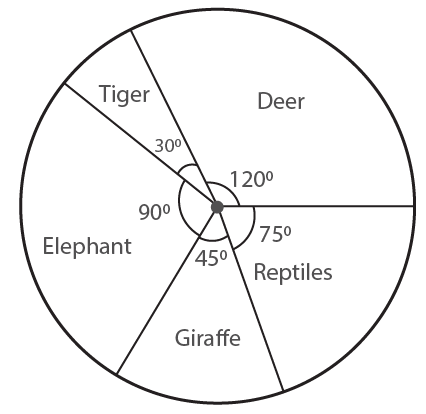2. The following data represents the monthly expenditure of a family (in T) on various items. Draw a pie chart to represent this data.

 Items Food House rent Education Savings Health Others Expenditure (in Rs) 12500 5000 7500 10000 5000 10000

Solution:

 Items Expenditure (in Rs) Central angles Food House rent Education Savings Health Others 12500 5000 7500 10000 5000 10000 (12500 × 3600) / 50000 = 900 (5000 × 3600) / 50000 = 360 (7500 × 3600) / 50000 = 540 (10000 × 3600) / 50000 = 720 (5000 × 3600) / 50000 = 360 (10000 × 3600) / 50000 = 720 Total 50000 3600

Pie chart for the given data is shown below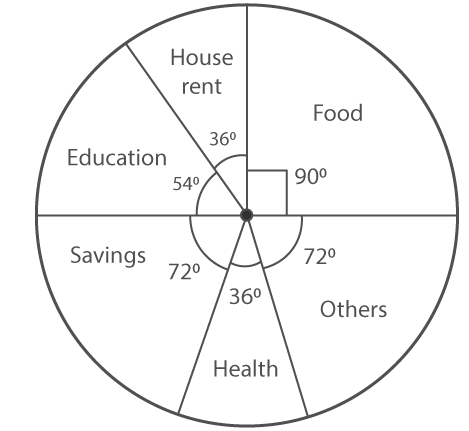3. The following data represents the percentage distribution of the expenditure incurred in publishing a book.

 Items Paper cost Printing cost Binding Royality Transportation cost Promotion cost Expenditure (in %) 25% 20% 20% 10% 15% 10%

Solution:

 Items Expenditure Central angles Paper cost Printing cost Binding Royality Transportation cost Promotion cost 25% 20% 20% 10% 15% 10% (3600 × 25) / 100 = 900 (3600 × 20) / 100 = 720 (3600 × 20) / 100 = 720 (3600 × 10) / 100 = 360 (3600 × 15) / 100 = 540 (3600 × 10) / 100 = 360 Total 100% 3600

Pie chart representing the given data is as follows: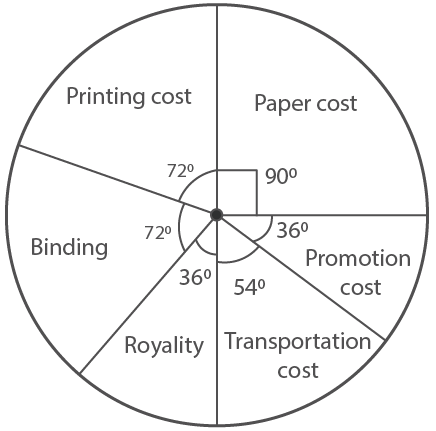4. The following data represents the number of students got admission in different streams of a college:

 Stream Science Arts Commerce Law Management Number of students 400 300 500 250 350

Draw a pie chart to represent this data

Solution:

 Stream Number of students Central angle Science Arts Commerce Law Management 400 300 500 250 350 (400 × 3600) / 1800 = 800 (300 × 3600) / 1800 = 600 (500 × 3600) / 1800 = 1000 (250 × 3600) / 1800 = 500 (350 × 3600) / 1800 = 700 Total 1800 3600

Pie chart representing the above given data is shown below: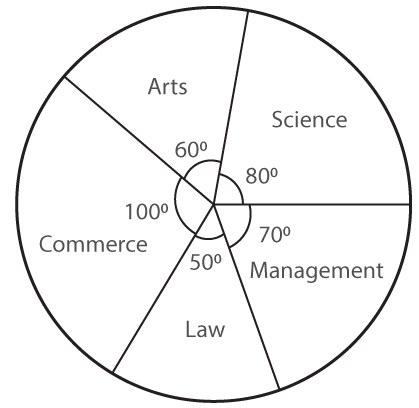5. The adjoining pie chart shows the expenditure of a country on various sports during year 2012. Study the pie chart carefully and answer the following questions: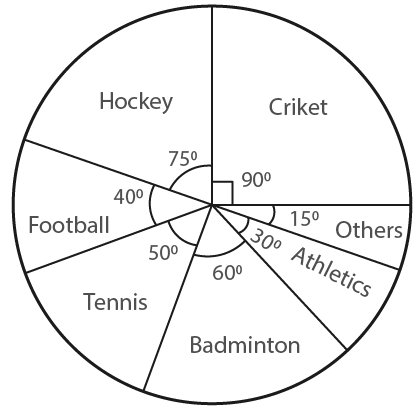(i) What percent of total expenditure is spent on cricket?

(ii) How much percent more is spent on hockey than that on tennis?

(iii) If the total amount spent on sports in 2012 is Rs 1, 80, 00, 000 then find the amount spent on Badminton

(iv) If the total amount spent on sports in 2012 is Rs 2, 40, 00, 000 then find the amount spent on cricket and hockey together.

Solution:

The given pie chart represents the expenditure of a country on various sports during year 2012

(i) Given that expenditure on a cricket = 900

So,

(90 / 3600) × 100% = 25%

Therefore, 25% expenditure is spent on cricket

(ii) Given that expenditure on a hockey = 750

So,

(70 / 3600) × 100% = (125 / 6)% =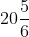%

Expenditure on tennis = 500

Hence, (50 / 3600) × 100% = (125 / 9)% = 13.9%

So,

(125 / 6) – (125 / 9) = 125 / 18

= (375 – 250) / 18

= 125 / 18

= 6.95% more

Therefore, 6.95% more is spent on hockey than that on tennis

(iii) Total amount spent on sports = Rs 1, 80, 00, 000

Total amount spent on Badminton = Rs 1, 80, 00,000 × (60 / 3600)

= Rs 30,00,000

(iv) If the total amount spent on sports = 2,40,00,000

Total amount spent on cricket and hockey together = 900 + 750

= 1650

= (1650 / 3600) × 2, 40, 00,000

= 1, 10, 00, 000

6. The adjoining pie chart shows the number of students enrolled in class VI to class X of a school.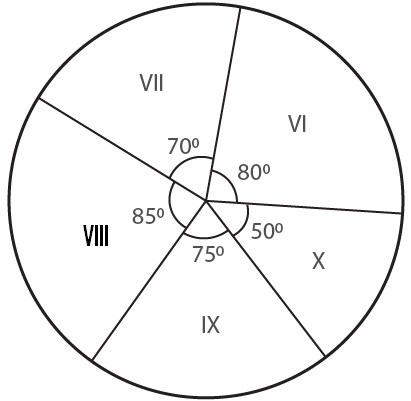If 1440 students are enrolled from VI to X, then answer the following questions:

(i) How many students are enrolled in class VIII?

(ii) How many students are more in class IX than in class X?

(iii) What is the sum of students enrolled in VII and VIII?

(iv) Find the ratio of students enrolled in VI to students enrolled in X

Solution:

The given pie chart represents the enrolment of students from class VI to class X in a school.

Total number of students enrolled from VI to X = 1440 students

(i) Enrolment of class VIII = (85 / 3600) × 1440 = 340 students

(ii) Difference in X and IX class enrolment = 750 – 500

= 250

(25 / 3600) × 1440 = 100 students

Therefore, 100 students are more in class IX than in class X

(iii) Sum of students enrolled in VII and VIII classes = 700 + 850

= 1550

(155 / 3600) × 1440 = 620 students

Therefore, the sum of students enrolled in class VI and VIII = 620 students

(iv) Ratio between the students enrolled in VI to students enrolled in X classes = 800: 500

= 8: 5

Therefore, the ratio between the students enrolled in VI to students enrolled in X classes is 8: 5

Exercise 19.3

1. List the outcomes you can see in these experiments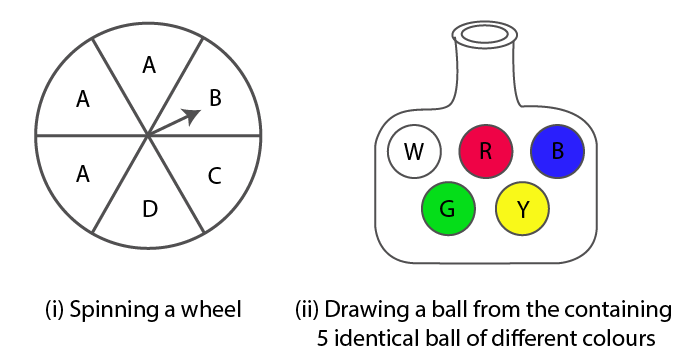Solution:

(i) The outcomes in spinning wheel = A, A, A, B, C, D

(ii) The outcomes in drawing a ball from a bag containing 5 identical balls of different colours = White, Red, Blue, Green, Yellow

2. A die is rolled once. Find the probability of getting

(i) an even number

(ii) a multiple of 3

(iii) not a multiple of 3

Solution:

Total outcomes of a die when rolled once:

1, 2, 3, 4, 5, 6 = 6

(i) An even number: 2, 4, 6

i.e, Favourable outcomes = 3

Therefore,

Probability P(E) = 3 / 6

= 1 / 2

(ii) Multiple of 3 = 3, 6

i.e, Favourable outcomes = 2

Therefore,

Probability P(E) = 2 / 6

= 1 / 3

(iii) Not a multiple of 3 = 1, 2, 4, 5

i.e Favourable outcomes = 4

Therefore,

Probability P(E) = 4 / 6

= 2 / 3

3. Two coins are tossed together. Find the probability of getting

(i) two tails

(ii) atleast one tail

(iii) no tail

Solution:

The total outcomes, when two coins are tossed together = 2 × 2

= 4

Therefore, outcomes are,

HH, HT, TH, TT

(i) Favourable outcomes of getting two tails = 1

Hence,

Probability P(E) = 1 / 4

(ii) Favourable outcomes of getting atleast one tail = TH, HT, TT

= 3

Hence,

Probability P(E) = 3 / 4

(iii) Favourable outcomes of getting no tail = HH

= 1

Hence,

Probability P(E) = 1 / 4

4. Three coins are tossed together. Find the probability of getting

(ii) atleast one tail

(iii) atmost one tail

Solution:

Three coins are tossed together

Hence,

Total outcomes = 8

= HHH, HHT, HTH, THH, HTT, TTH, TTT, THT

(i) Favourable outcomes of getting atleast two heads = HHH, HHT, HTH, THH

= 4 in numbers

Therefore,

Probability P(E) = (Number of favourable outcome) / (Number of possible outcome)

= 4 / 8

= 1 / 2

(ii) Favourable outcomes of getting atleast one tail = HHT, HTH, HTT, TTT, THH, THT, TTH

= 7 in numbers

Therefore,

Probability P(E) = (Number of favourable outcome) / (Number of possible outcome)

= 7 / 8

(iii) Favorable outcomes of getting atmost one tail = HHH, HHT, HTH, THH

= 4 in numbers

Therefore,

Probability P(E) = (Number of favourable outcome) / (Number of possible outcome)

= 4 / 8

= 1 / 2

5. Two dice are rolled simultaneaously. Find the probability of getting

(i) the sum as 7

(ii) the sum as 3 or 4

(iii) prime numbers on both the dice.

Solution:

Two dice are rolled simultaneously, then

Total outcomes = 6 × 6

= 36

(i) Sum as 7 = (1, 6), (2, 5), (3, 4), (4, 3), (5, 2), (6, 1)

= 6

Therefore,

Probability P(E) = (Favourable outcome) / (Total outcome)

= 6 / 36

= 1 / 6

(ii) The sum as 3 or 4 = (1, 2), (1, 3), (2, 1), (2, 2), (3, 1)

= 5

Therefore,

Probability P(E) = (Favourable outcome) / (Total outcome)

= 5 / 36

(iii) Prime numbers on both the side = (2, 2), (2, 3), (2, 5), (3, 2), (3, 3), (3, 5), (5, 2), (5, 3), (5, 5)

= 9

Therefore,

Probability P(E) = (Favourable outcome) / (Total outcome)

= 9 / 36

= 1 / 4

6. A box contains 600 screws, one tenth are rusted. One screw is taken out at random from the box. Find the probability that it is

(i) a rusted screw

(ii) not a rusted screw

Solution:

Given

Rusted screw = (1 / 10) of 600

= (1 / 10) × 600

= 60 seconds

(i) Favourable outcomes of picking rusted screw = 60

Therefore,

Probability P(E) = 60 / 600

= 1 / 10

(ii) Probability (of not rusted screw) = 1 – Probability (of rusted screw)

= 1 – 1 / 10

= (10 – 1) / 10

= 9 / 10

7. A letter is chosen from the word ‘TRIANGLE’. What is the probability that it is a vowel?

Solution:

Given word,

‘TRIANGLE’

Total number of outcomes = 8

Vowels = I, A, E = 3

Therefore,

Probability P(E) = 3 / 8

Hence, the probability of vowel in ‘TRIANGLE’ is 3 / 8

8. A bag contains 5 red, 6 black and 4 white balls. A ball is drawn at random from the bag, find the probability the ball is drawn is

(i) white

(ii) not black

(iii) red or black

(iv) neither red nor black

Solution:

Given

In a bag, there are 5 red, 6 black and 4 white balls.

Then, total number of outcomes = 5 + 6 + 4

= 15

(i) Probability of white ball = 4 / 15

(ii) Probability of not black = 5 + 4

= 9 balls

Therefore,

Probability of not black = 9 / 15 = 3 / 5

(iii) Probability of red or black = 5 + 6

= 11

Therefore,

Probability of red or black = 11 / 15

(iv) Probability of ball which is neither red nor black, i.e, white ball = 4

Therefore,

Probability of ball which is neither red nor black = 4 / 15

9. A box contains 17 cards numbered 1, 2, 3…….., 17 and are mixed thoroughly. A card is drawn at random from the box. Find the probability that the number on that card is

(i) odd

(ii) even

(iii) prime

(iv) divisible by 3

(v) divisible by 2 and 3 both

Solution:

Given

A box contains 17 cards numbered 1 to 17

So, total number of outcomes = 17

(i) Card bearing odd number

(1, 3, 5, 7, 9, 11, 13, 15, 17) = 9

Therefore,

Probability P(E) = 9 / 17

(ii) Even number

(2, 4, 6, 8, 10, 12, 14, 16) = 8

Therefore,

Probability P(E) = 8 / 17

(iii) Prime numbers

(2, 3, 5, 7, 11, 13, 17) = 7

Therefore,

Probability P(E) = 7 / 17

(iv) Numbers divisible by 3

3, 6, 9, 12, 15 = 5

Therefore,

Probability P(E) = 5 / 17

(v) Numbers divisible by 2 and 3 both

6, 12 = 2

Therefore,

Probability P(E) = 2 / 17

10. A card is drawn from a well-shuffled pack of 52 cards. Find the probability that the card drawn is:

(i) an ace

(ii) a red card

(iii) neither a king nor a queen

(iv) a red face card or an ace

(vi) non-face card of red colour

Solution:

Total number of playing cards = 52

One card is drawn

(i) An ace = 4

Therefore,

Probability P(E) = 4 / 52

= 1 / 13

(ii) A red card = 13 + 13 = 26

Therefore,

Probability P(E) = 26 / 52

= 1 / 2

(iii) Neither a king nor a queen

Number of cards = 52 – (4 + 4)

= 52 – 8

= 44

Therefore,

Probability P(E) = 44 / 52

= 11 / 13

(iv) A red face card = 6

Therefore,

Probability P(E) = 6 / 52

= 3 / 26

(v) A card of spade or an ace = 13 + 3

= 16

Therefore,

Probability P(E) = 16 / 52

= 4 / 13

(vi) Non-face card of red colour = 26 – 6

= 20

Therefore,

Probability P(E) = 20 / 52

= 5 / 13

11. In a lottery, there are 5 prized tickets and 995 blank tickets. A person buys a lottery ticket. Find the probability of his winning a prize.

Solution:

Given

Number of prized tickets = 5

Number of blank tickets = 995

So, total number of tickets = 5 + 995

= 1000

Probability of prized ticket P(E) = (Number of favourable outcome) / (Number of possible outcome)

= 5 / 1000

= 1 / 200

Therefore, the probability of his winning prize is 1 / 200